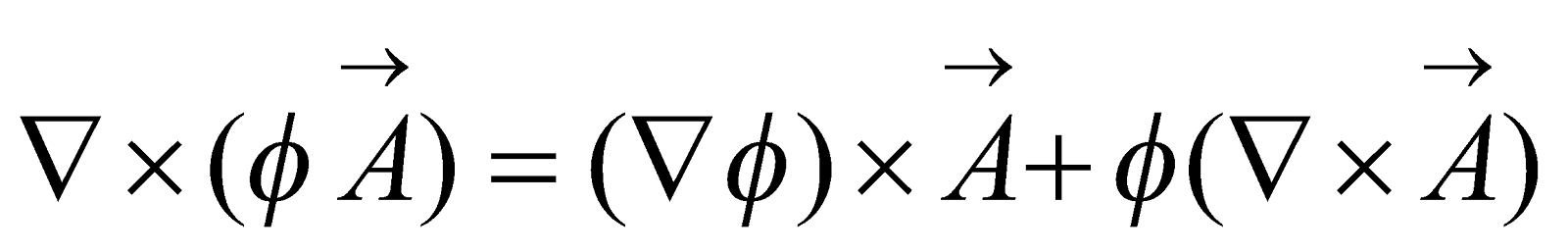## Friday, January 04, 2019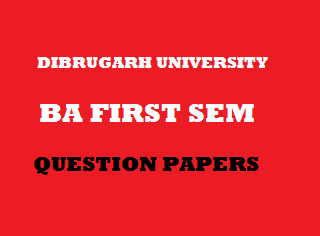2012
(November)
MATHEMATICS
(Major)
Course: 101
(Classical Algebra, Trigonometry and Vector Calculus)
Full Marks: 80
Pass Marks: 32
Time: 3 hours
The figures in the margin indicate full marks for the questions
GROUP – A
(Classical Algebra)

1. Answer the following questions: 1x4=4
1. Define a null sequence.
2. Give an example of an oscillatory series.
3. Write the necessary condition for convergence of an infinite series.
4. Write the equation whose roots are the roots of the following equation with opposite signs: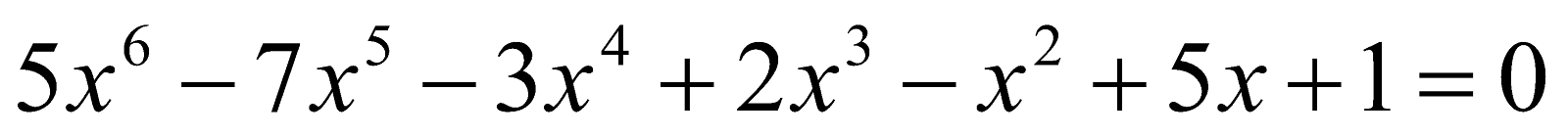1. Write the limit point(s) and the range set of the following sequence: 2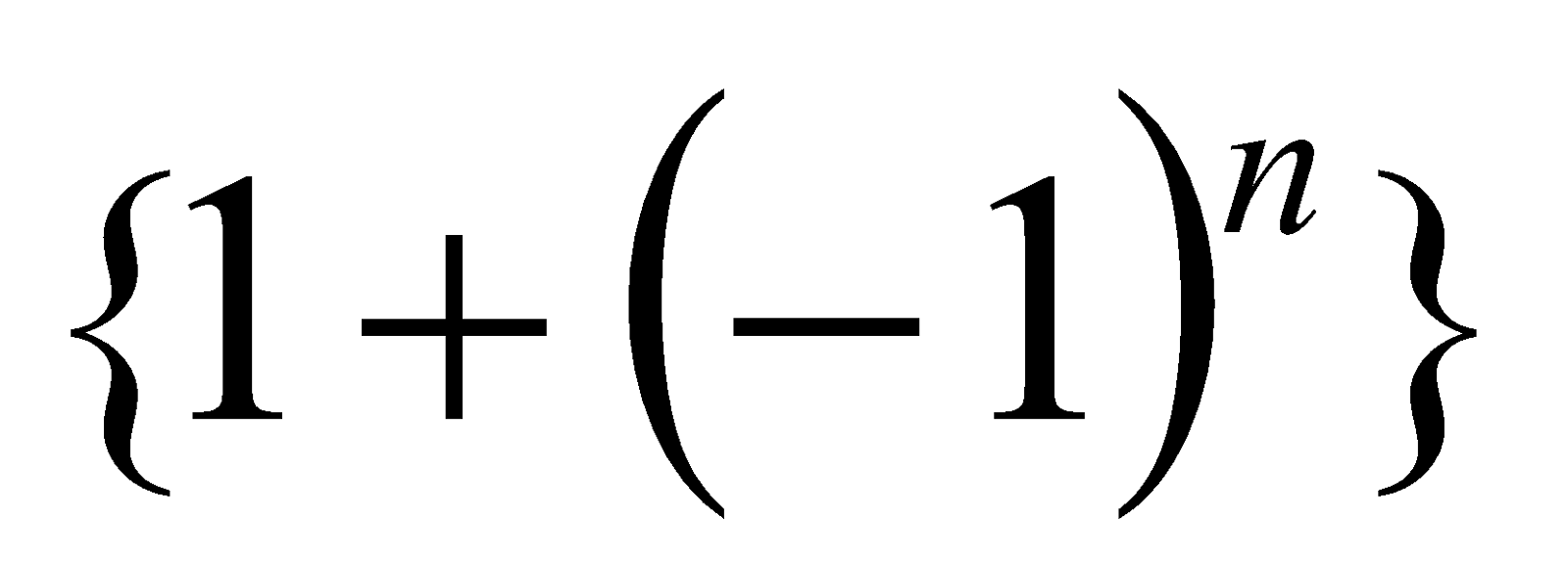Or
Show that the series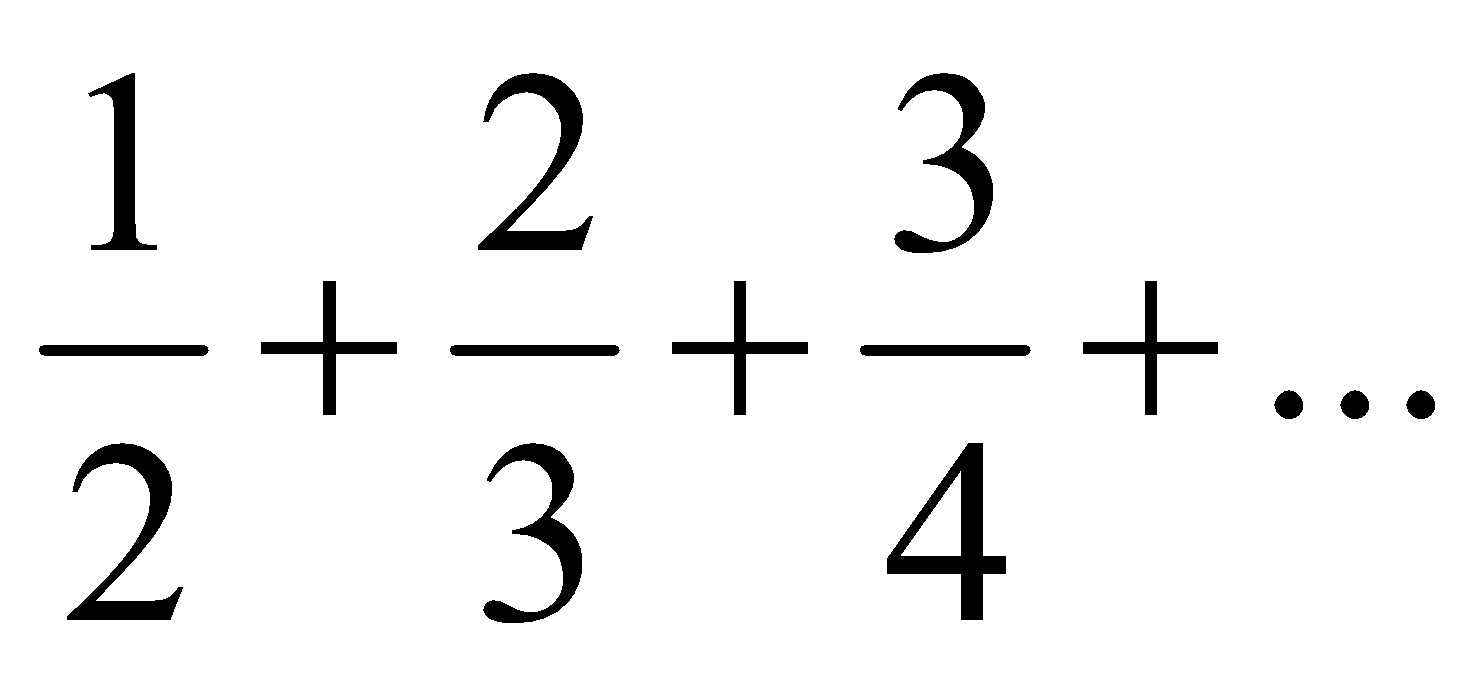does not converge.
1. Prove that every convergent sequence is bounded. Is the converse true? 2+1=3
2. Using comparison test, find whether the series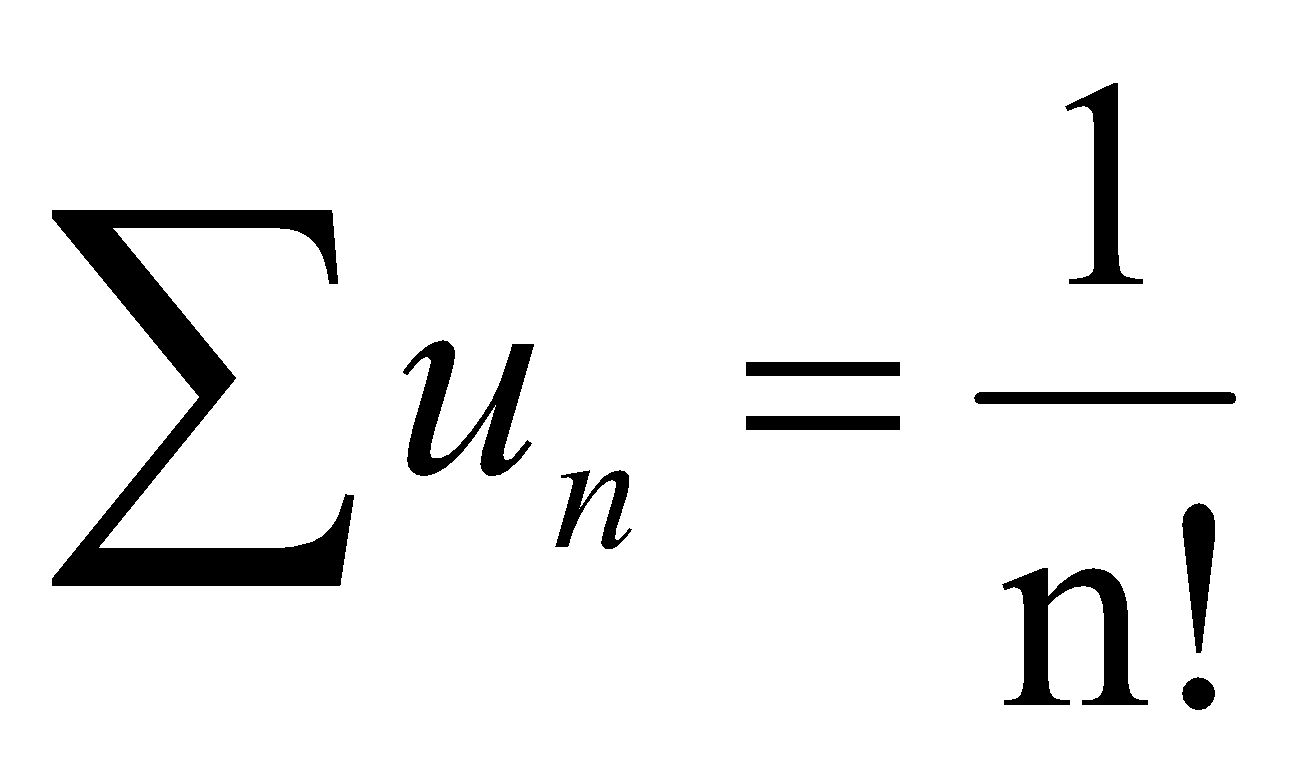is convergent. 3
Or
Using Leibnitz test for the convergence of alternating series, show that the series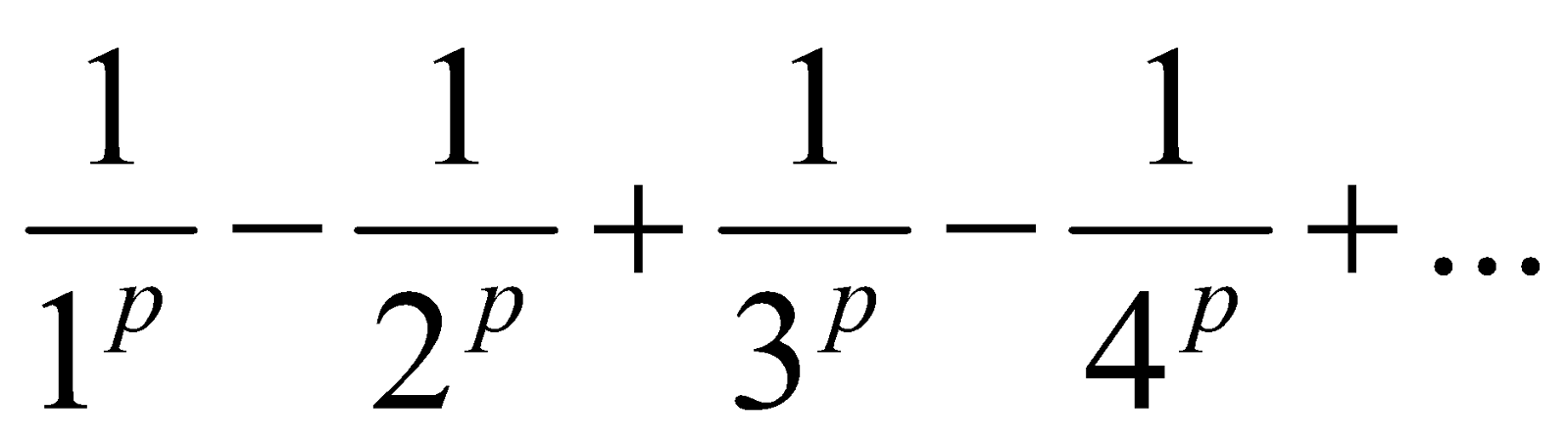Converges for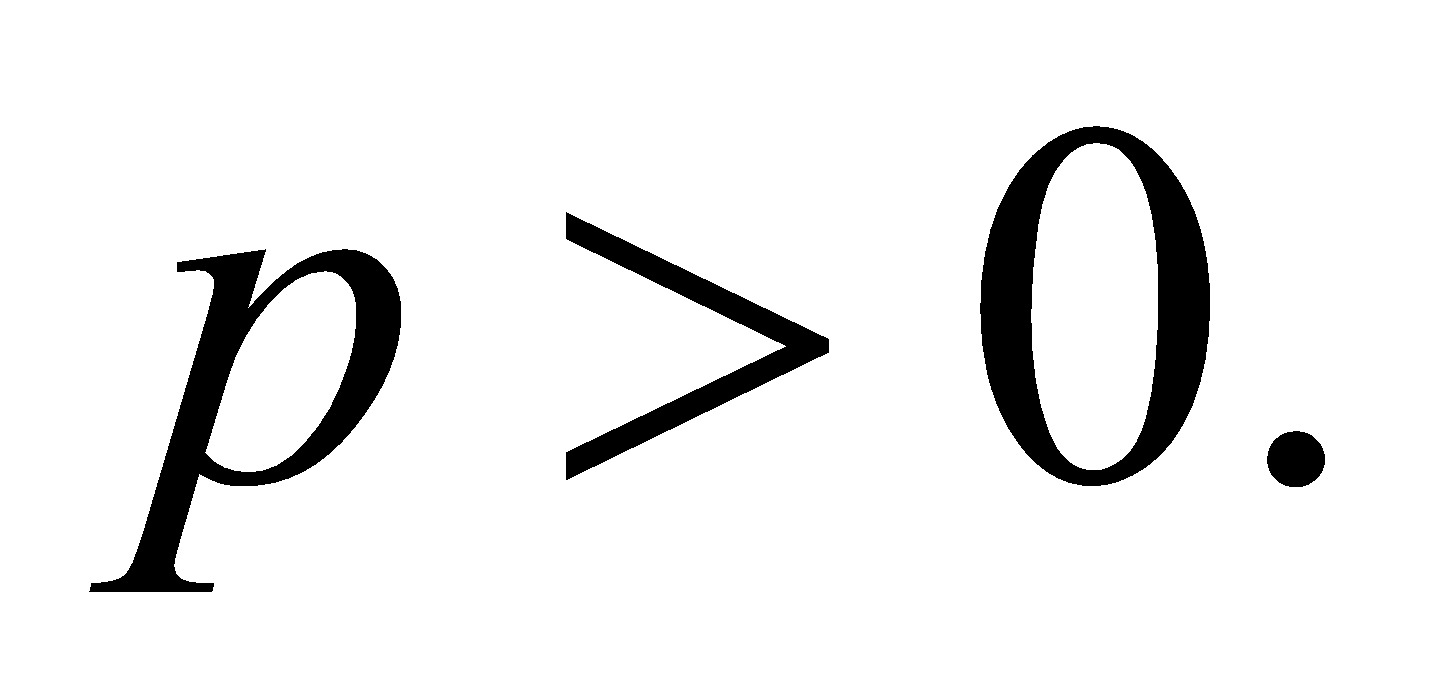3. (a) State and prove the factor theorem for polynomial equations. 4
(b) Show that the sequence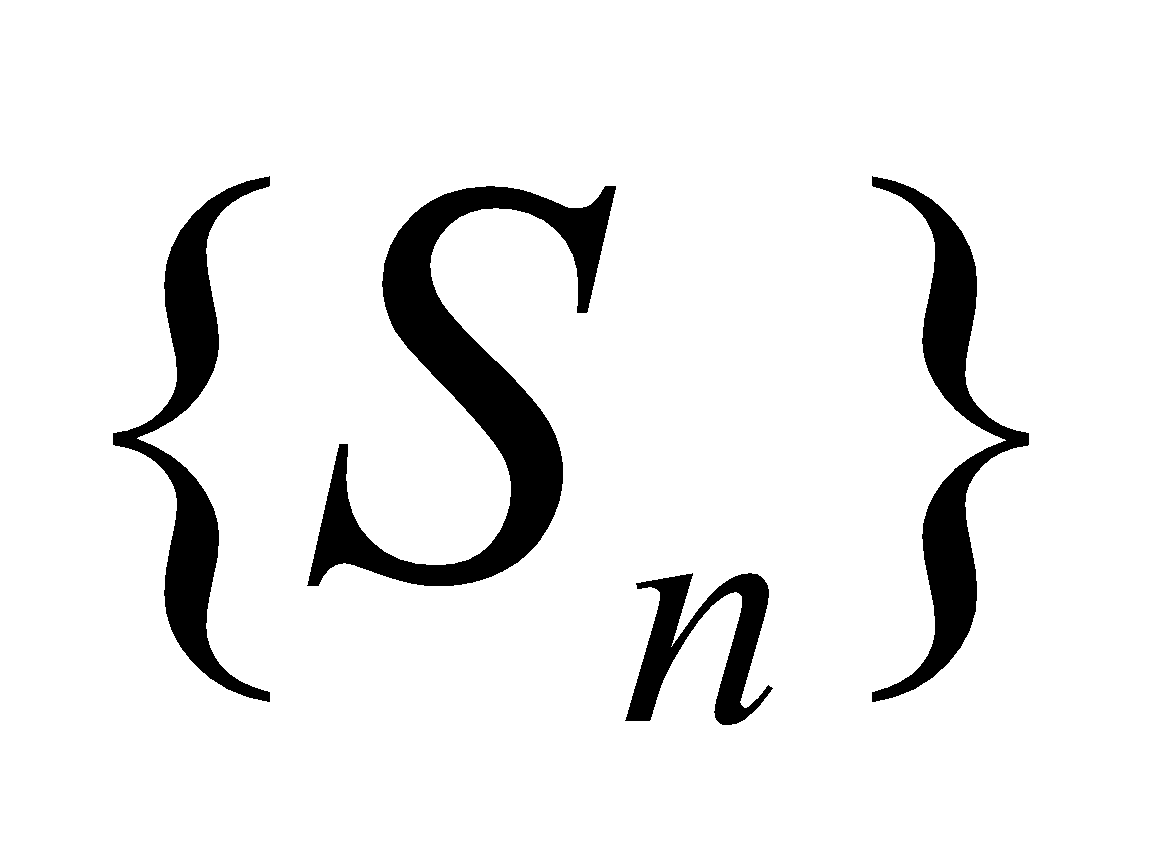, where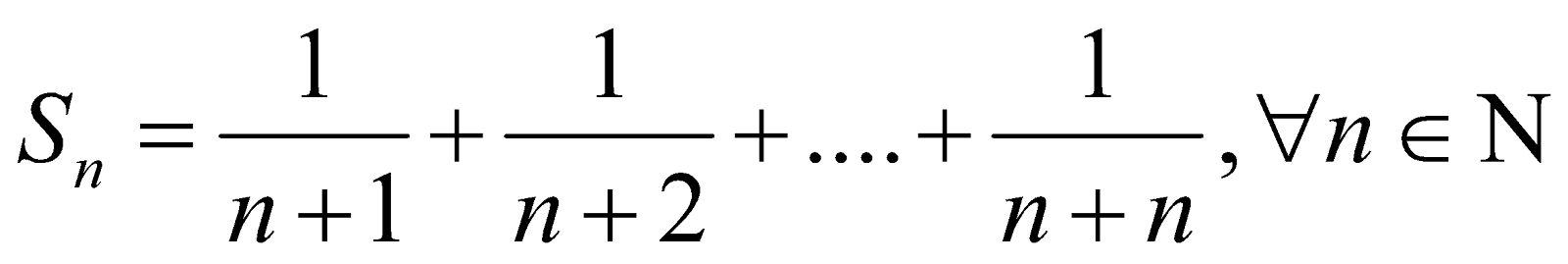is convergent. 4
(c) Test for convergence of the series 5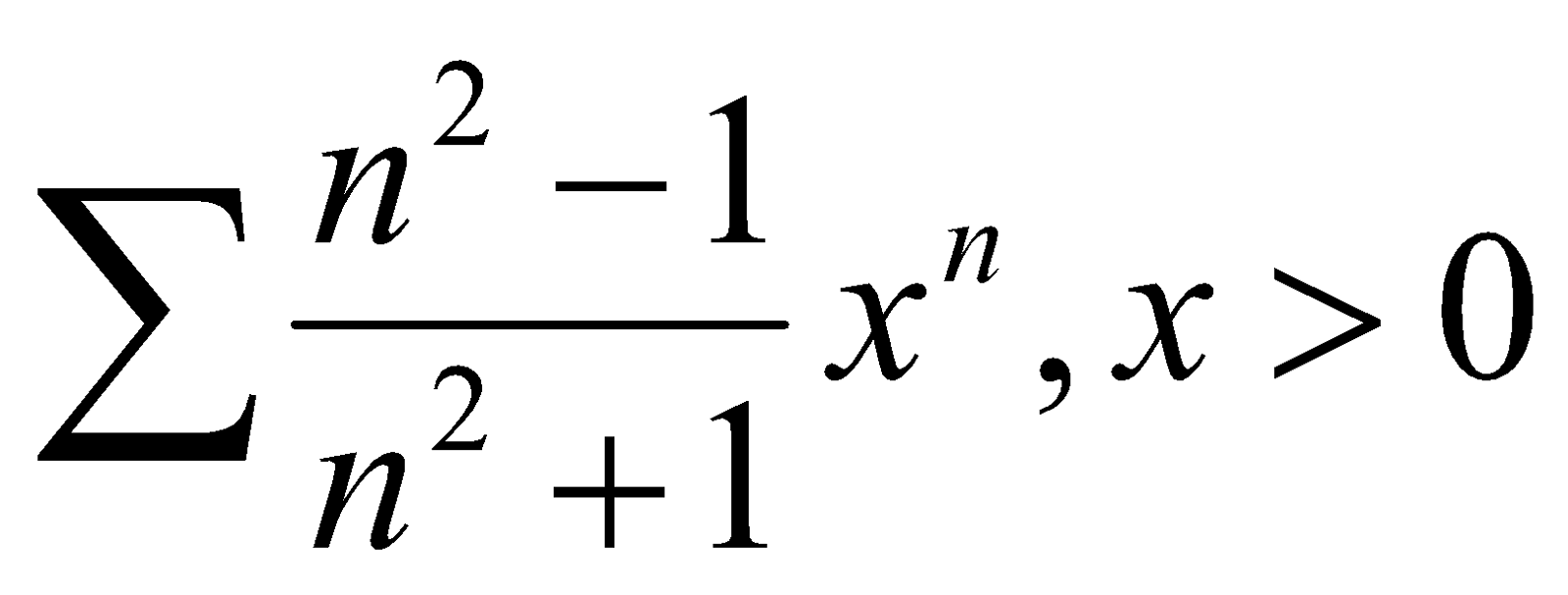(d) Test the convergence of the series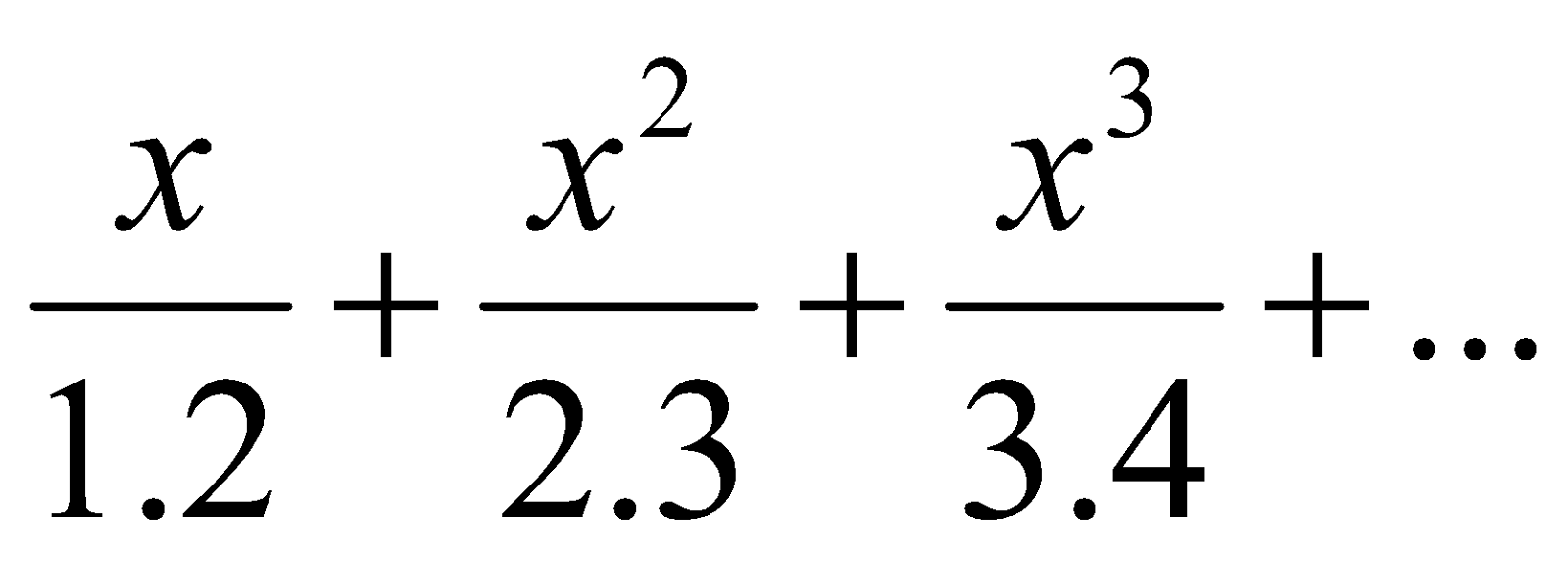5
4. (a) From the equation whose roots are the squares of the differences of the roots of the cubic equation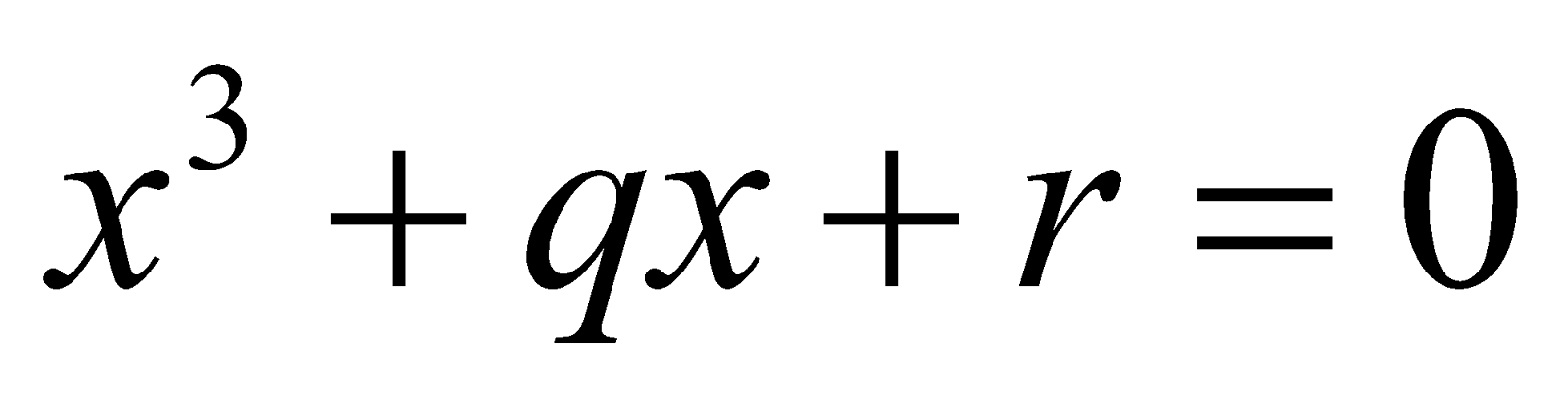5
Or
If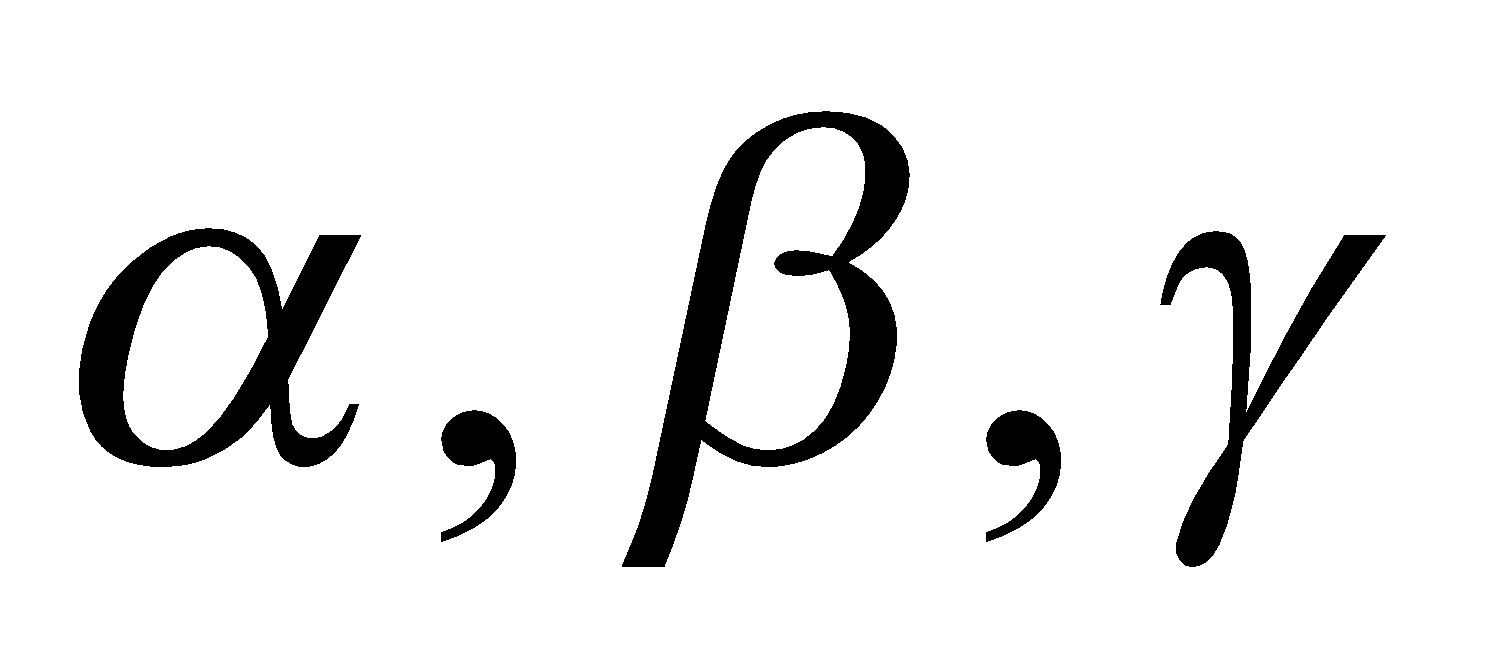are the roots of the equation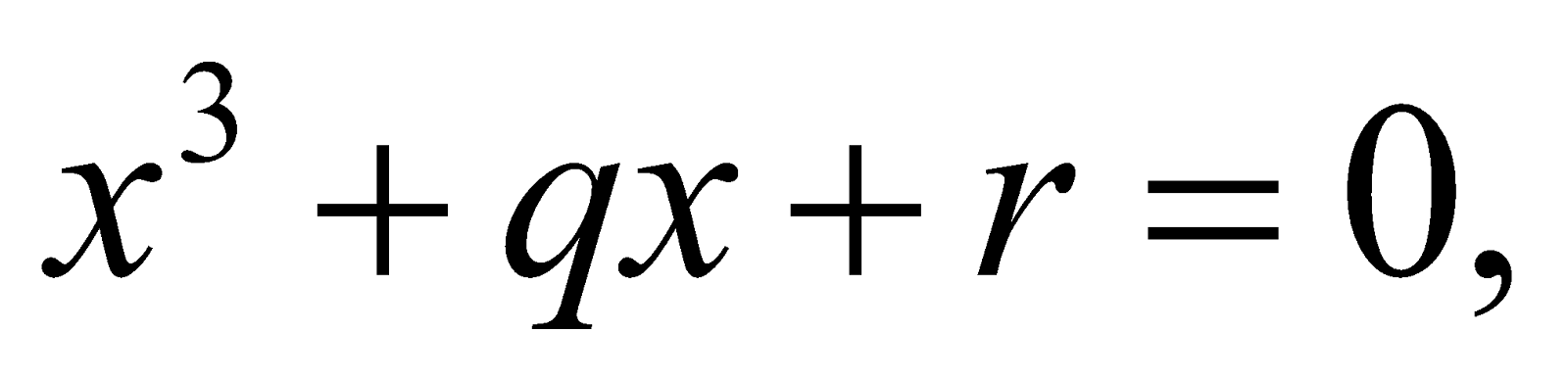then find the equation whose roots are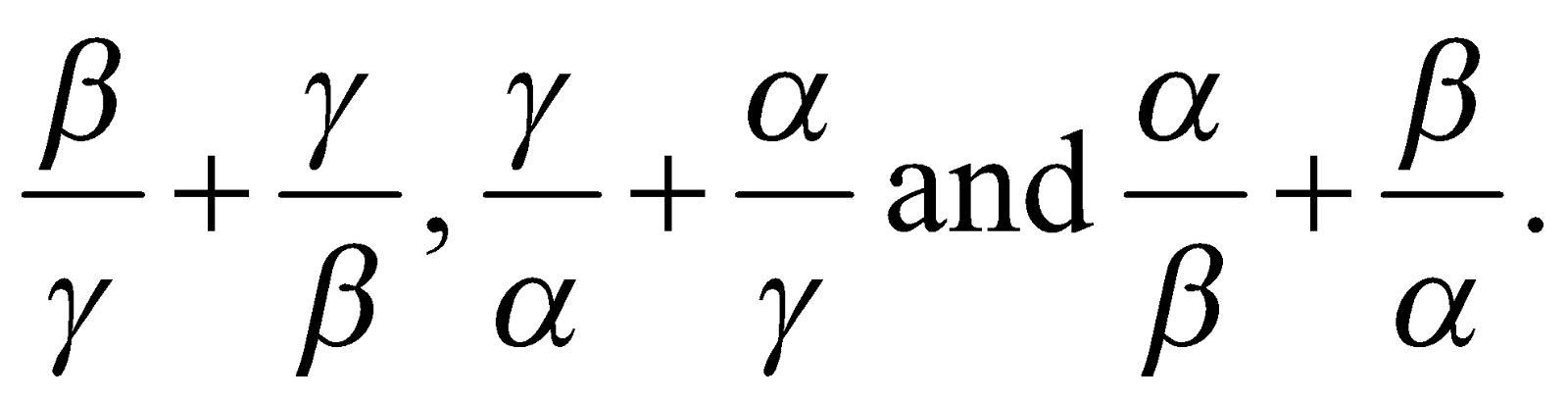(b) Discuss the Cardan’s method of solving a general cubic equation. 5
Or
Solve the equation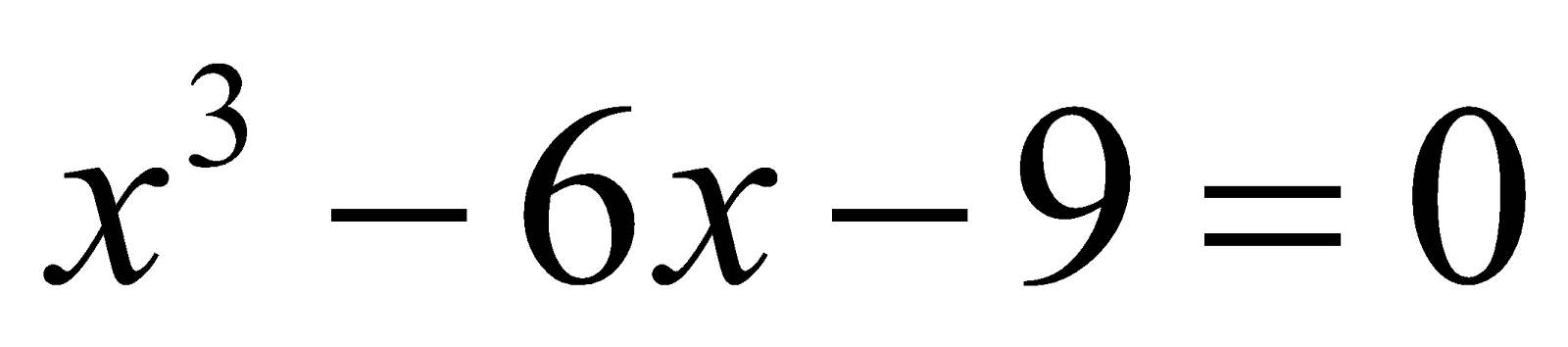by Cardan’s method.

GROUP – B
(Trigonometry)
5. (a) How many different values can be obtained for the following expression? 1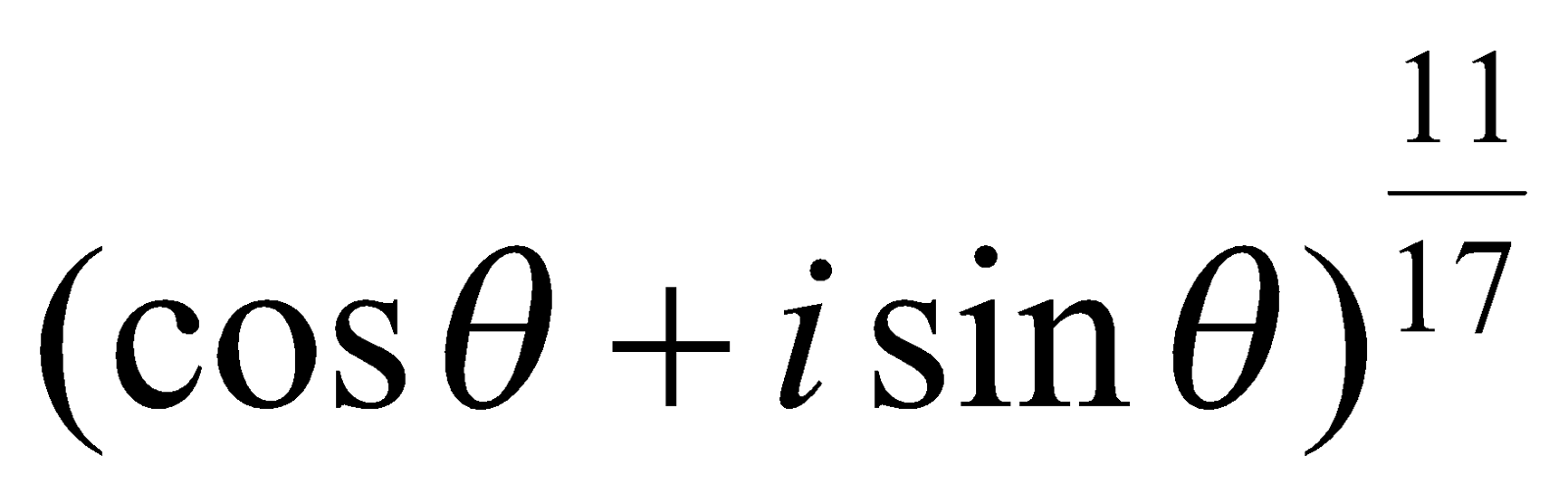(b) Distinguish between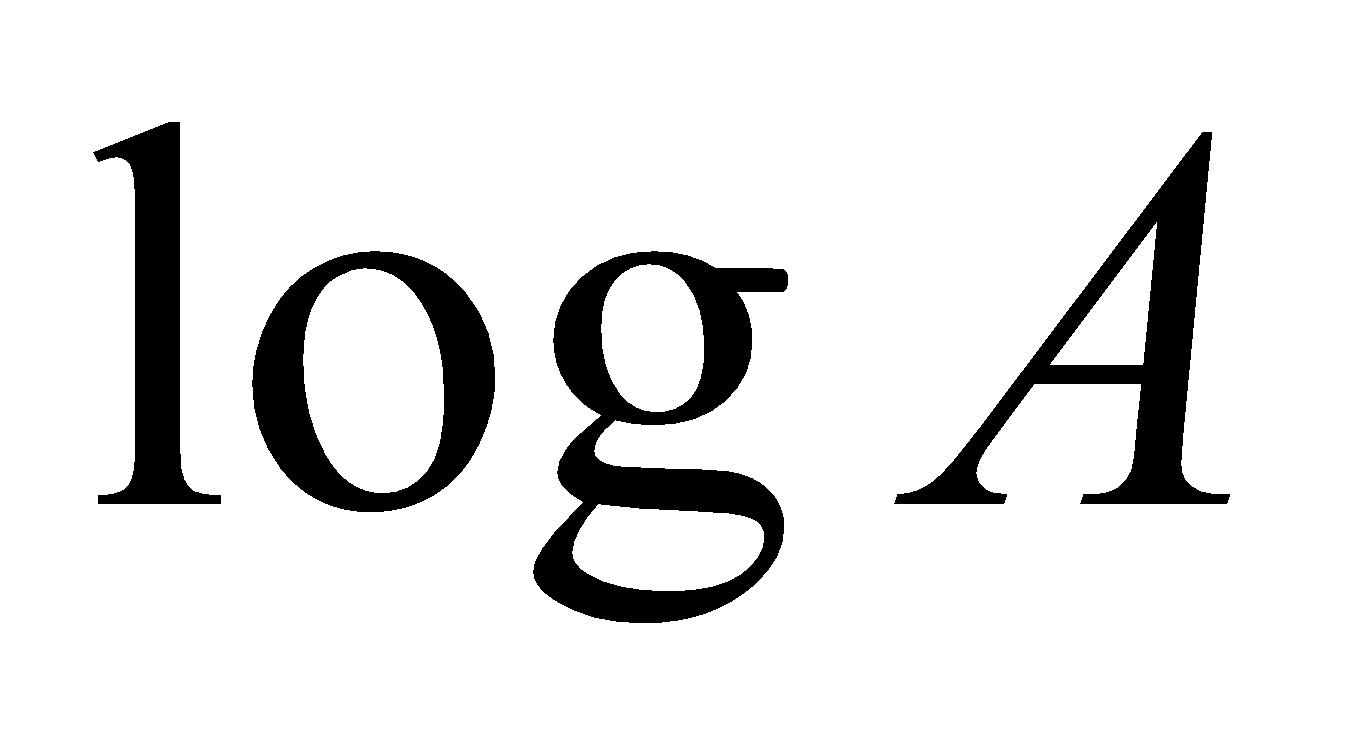andwhere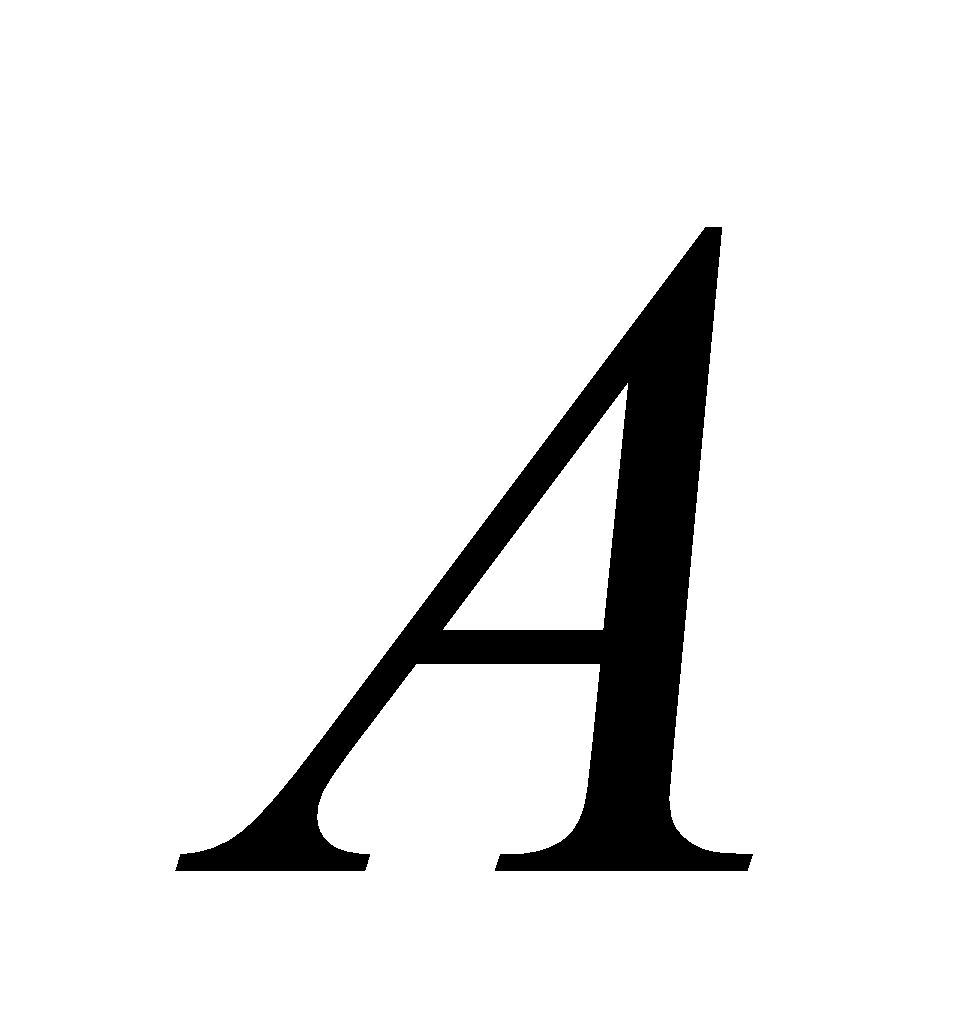is a complex quantity. 1
1. Express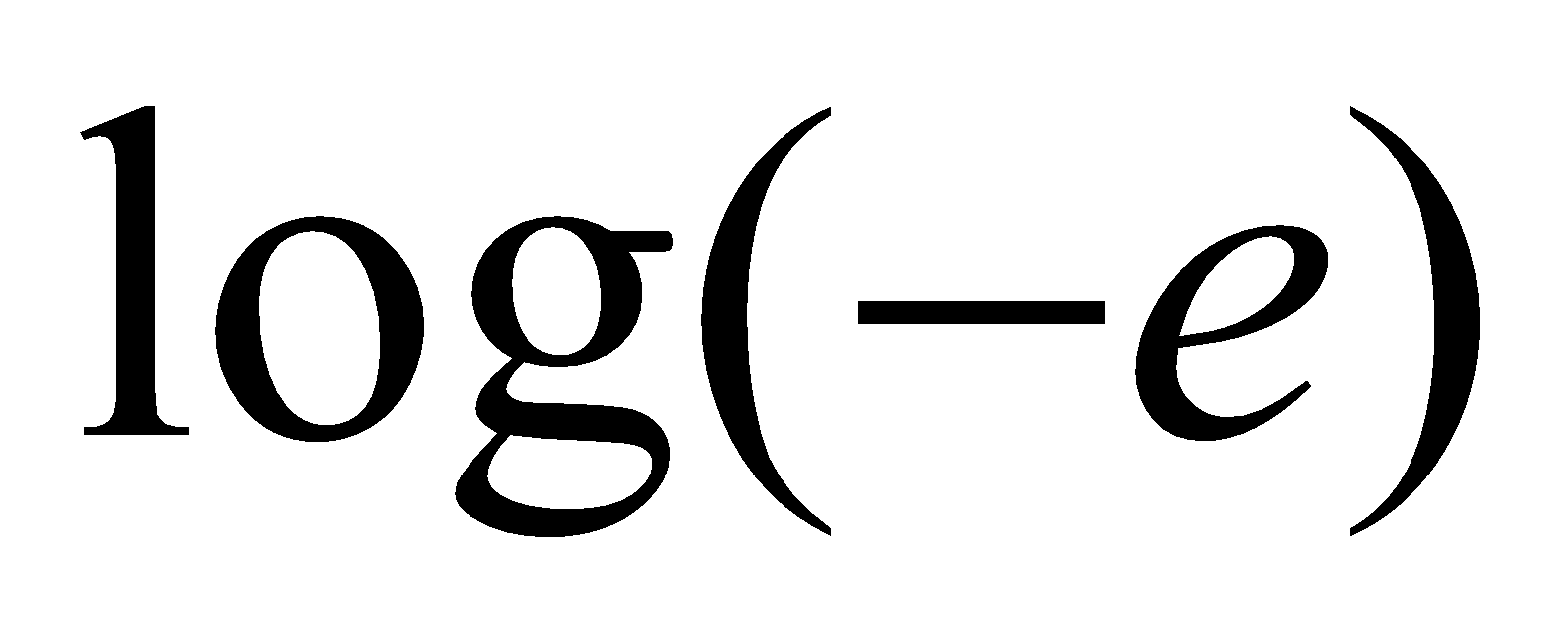in the form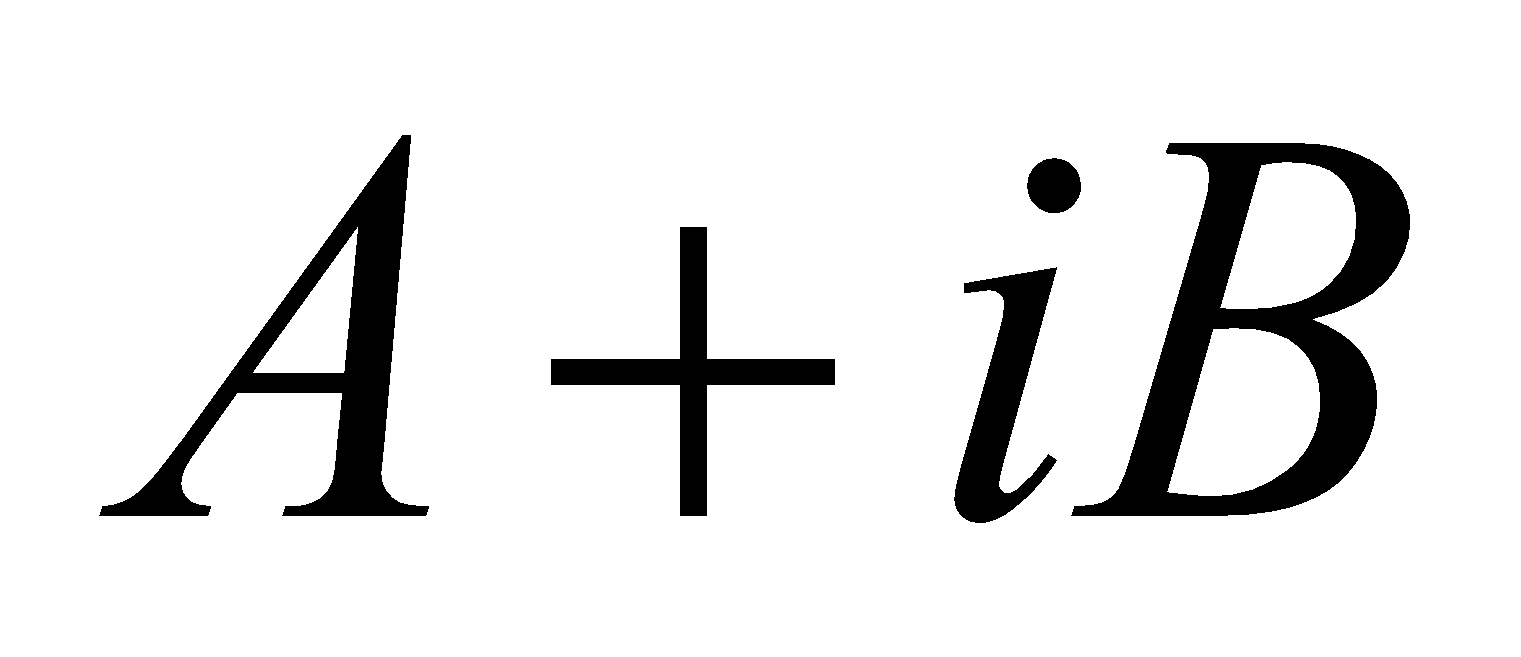.
2. Find the real part of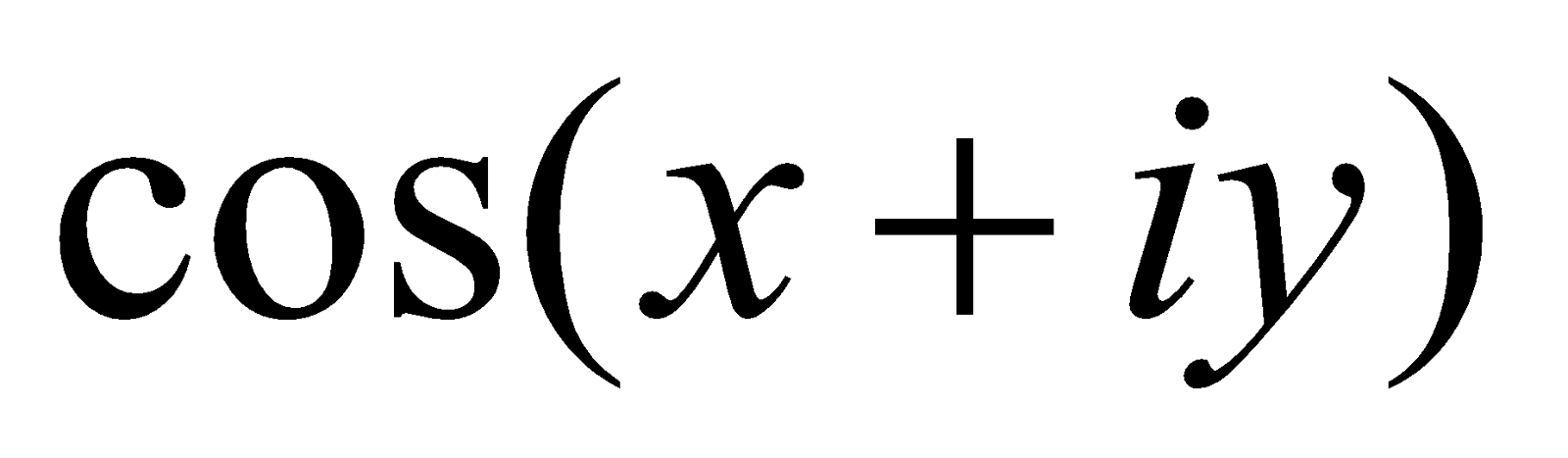.
3. Find all the values of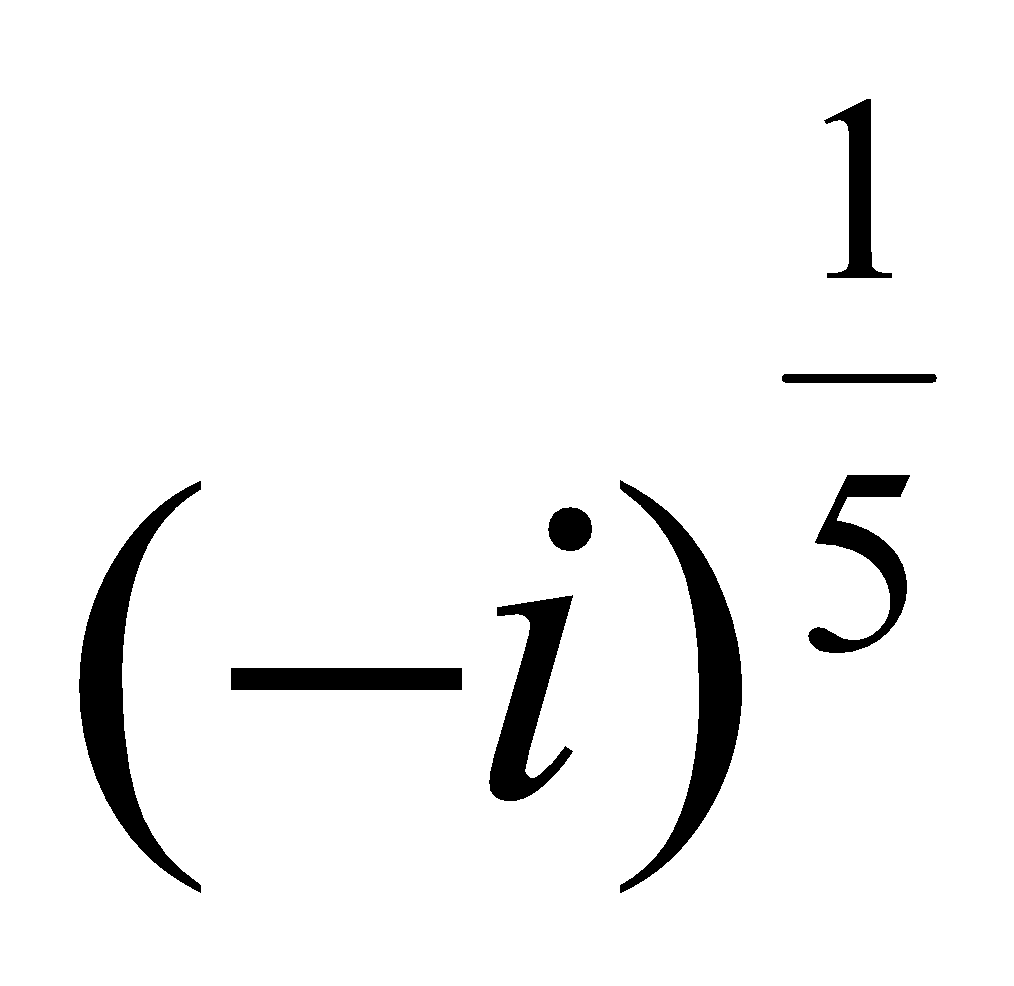.
7. (a) Using De Moivre’s theorem, prove that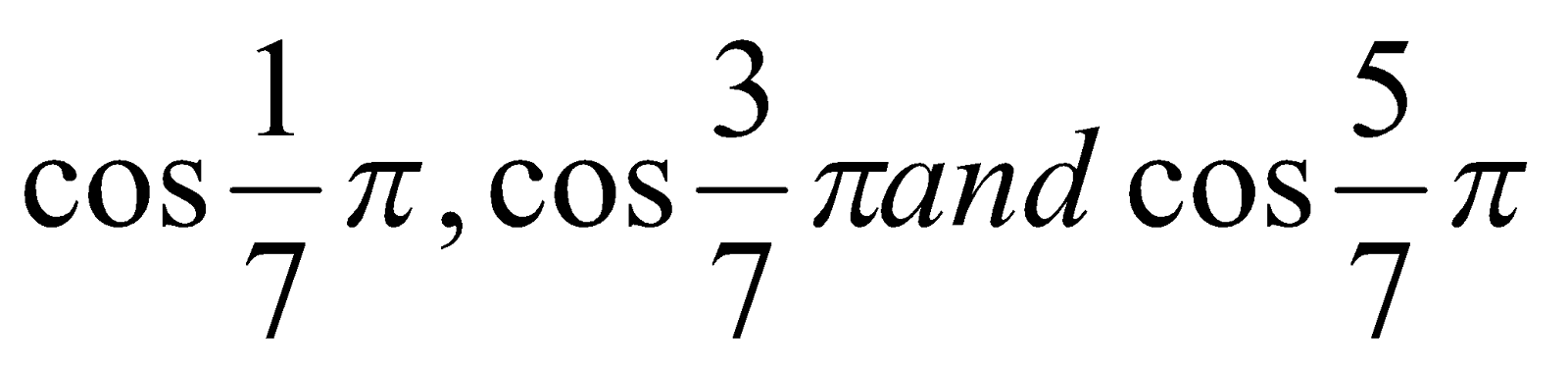are the roots of the cubic equation5
(b) Sum to n terms the series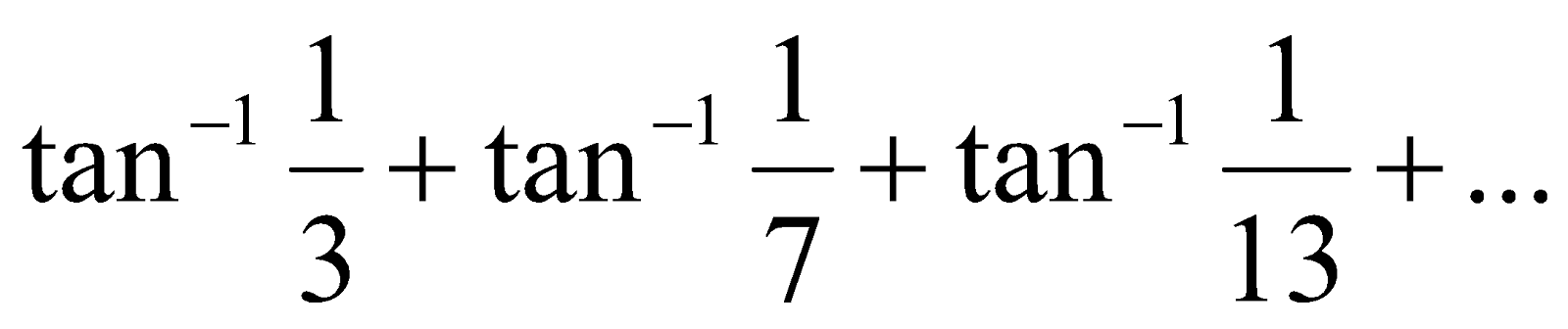4
(c) Expand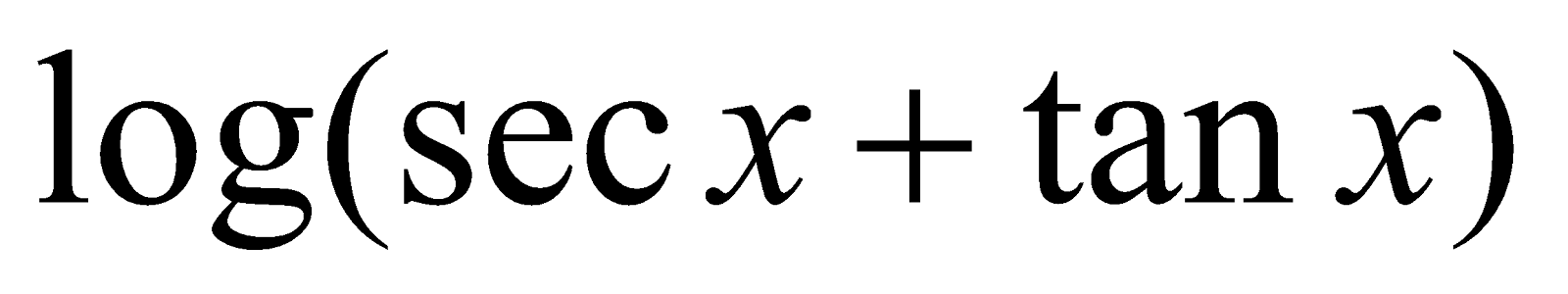in ascending powers of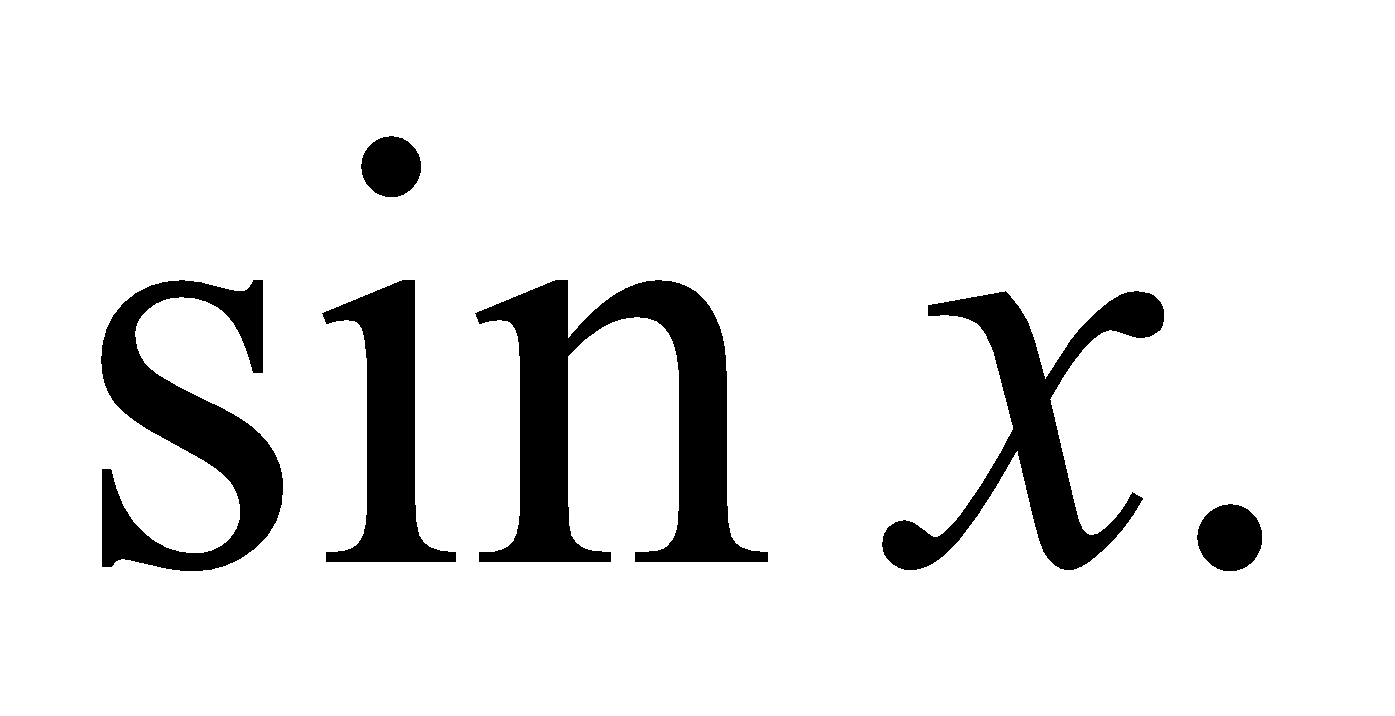(d) If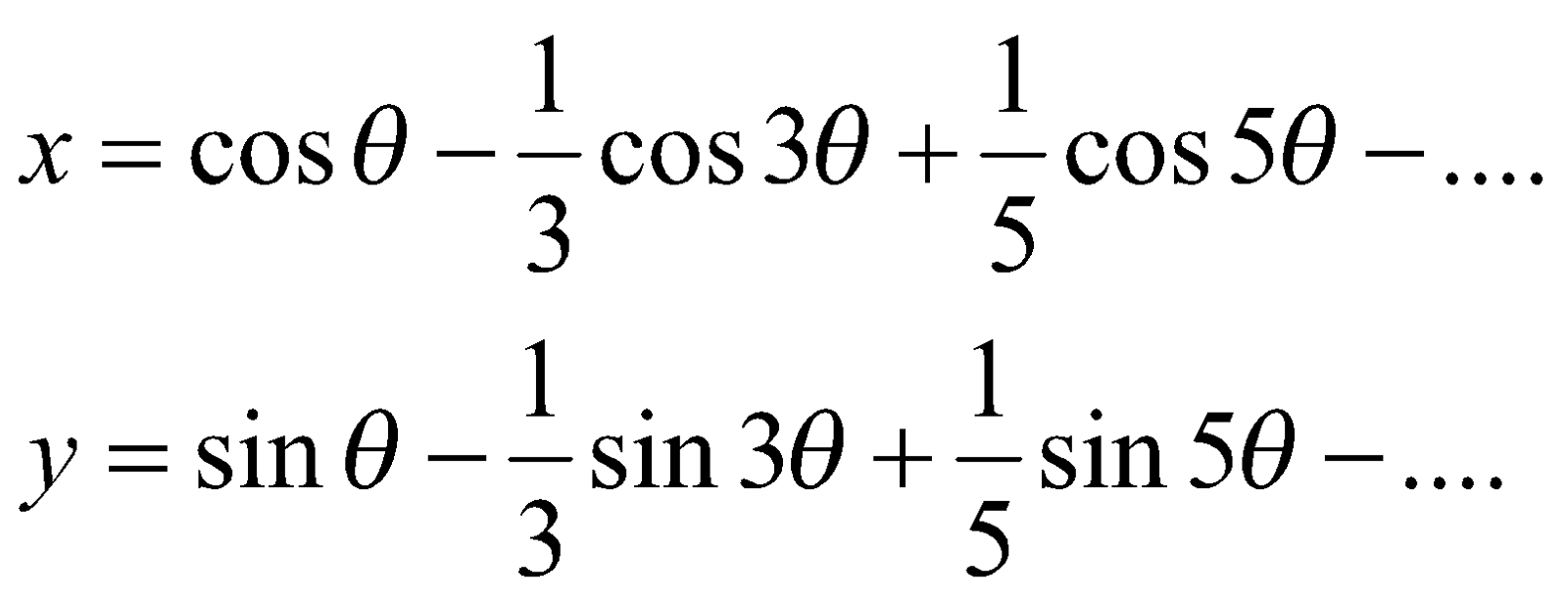then show that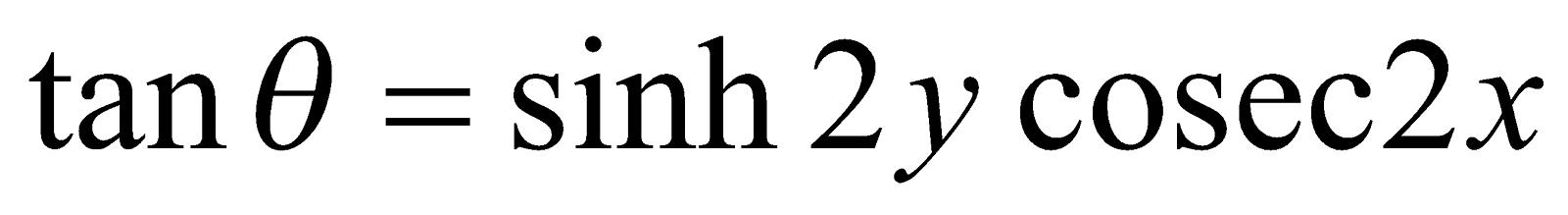5

GROUP – C
(Vector Calculus)
8. (a) What is the physical interpretation of directional derivative of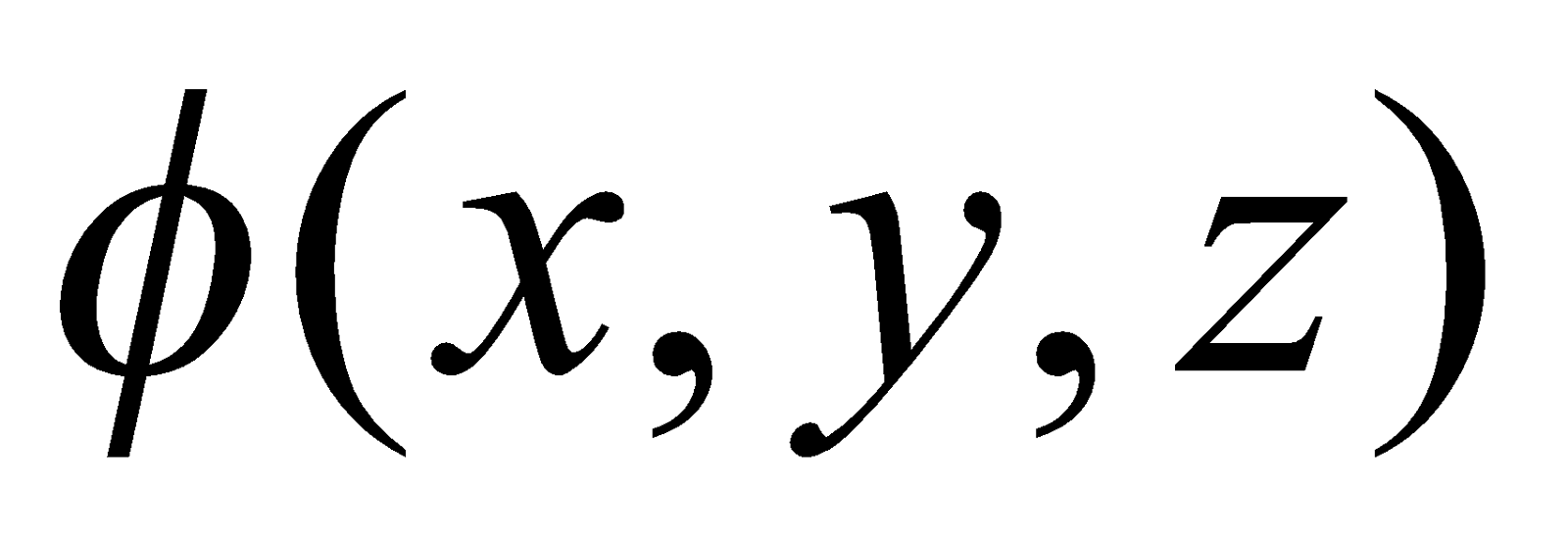in the direction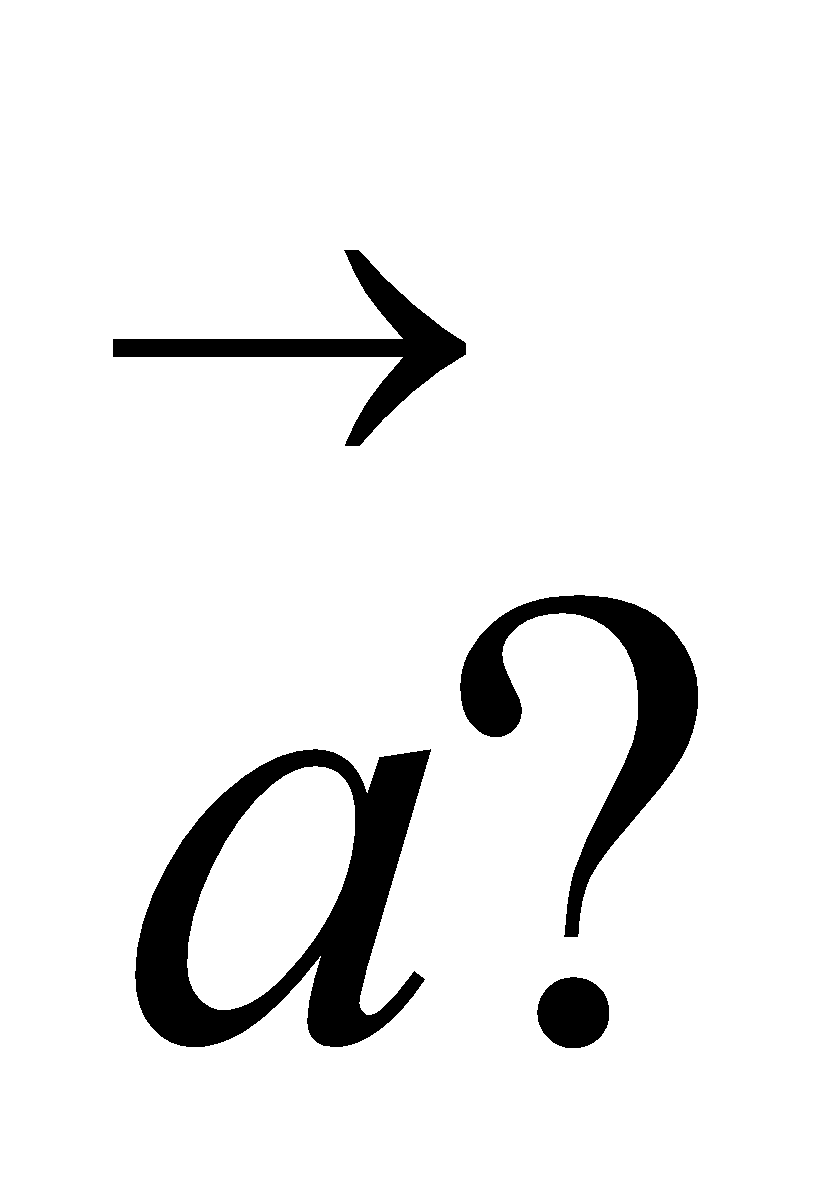(b) What do you mean by a solenoidal vector?
9. If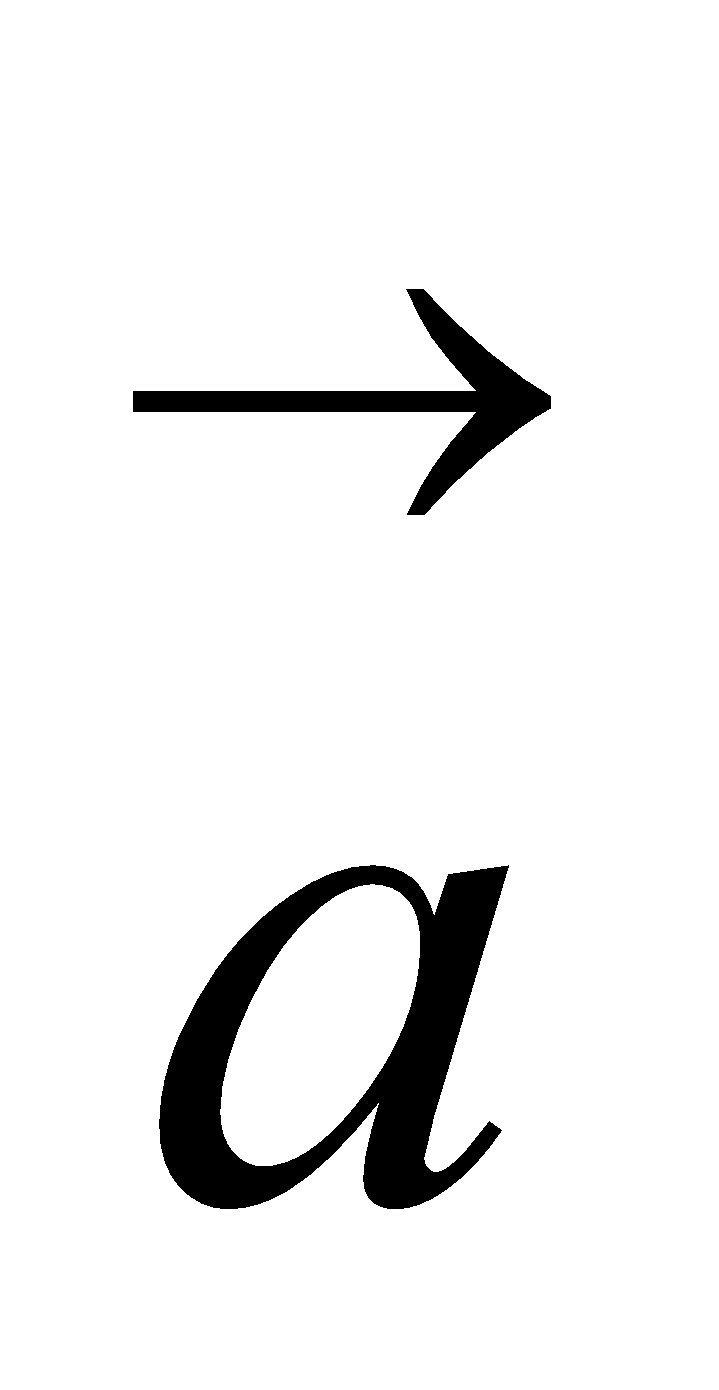has constant magnitude, then show thatand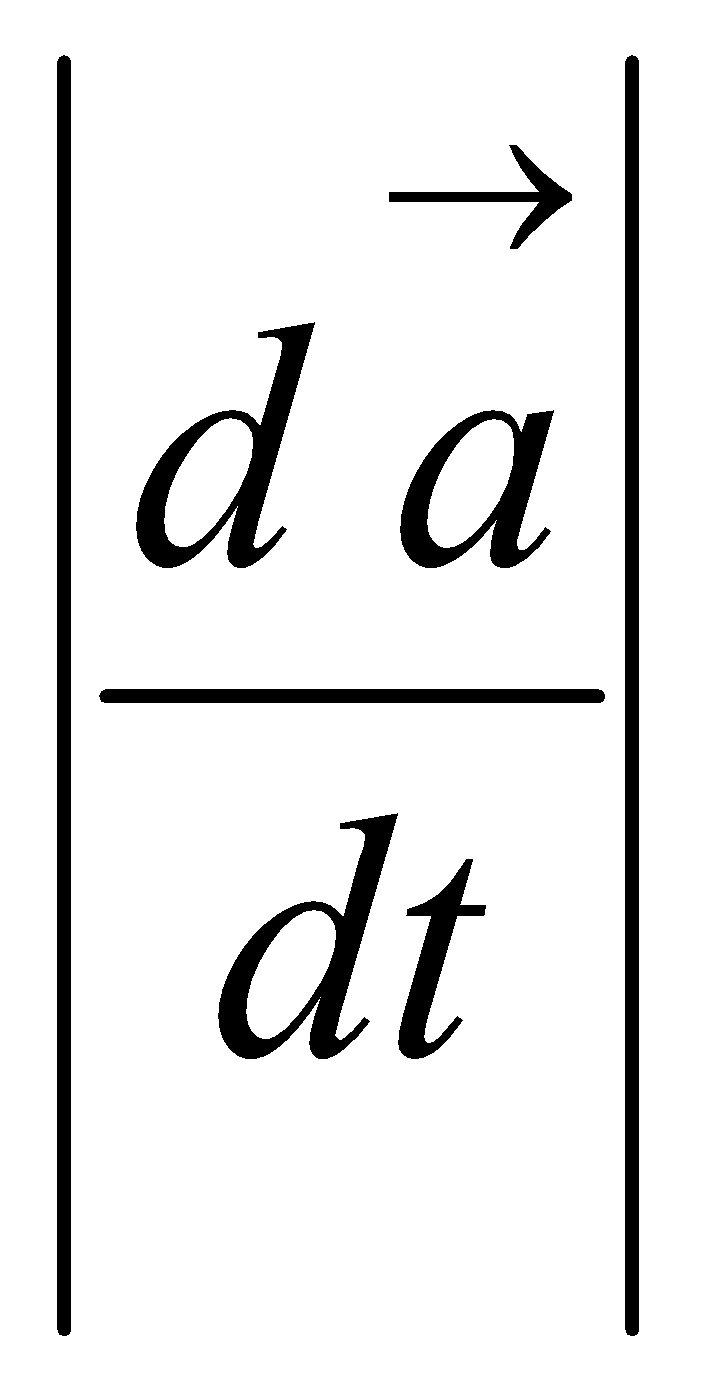are perpendicular to each other provided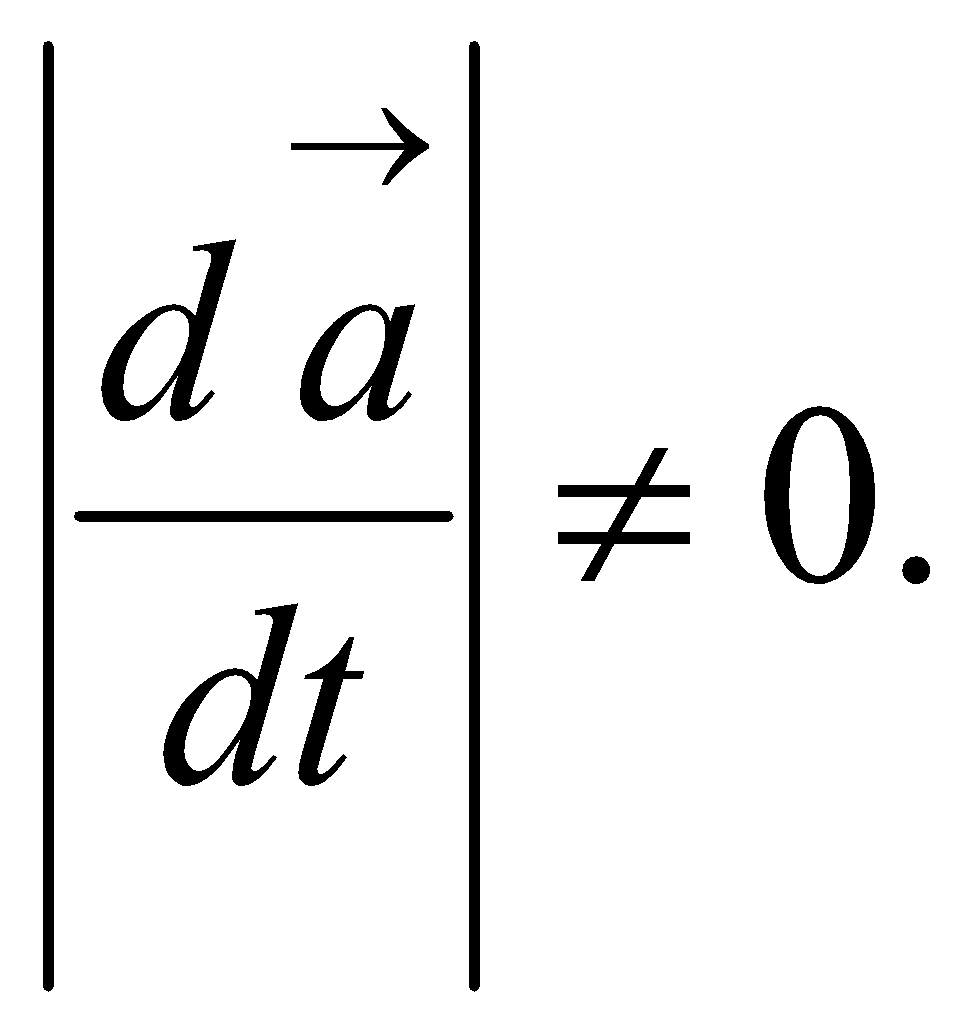Or
Show that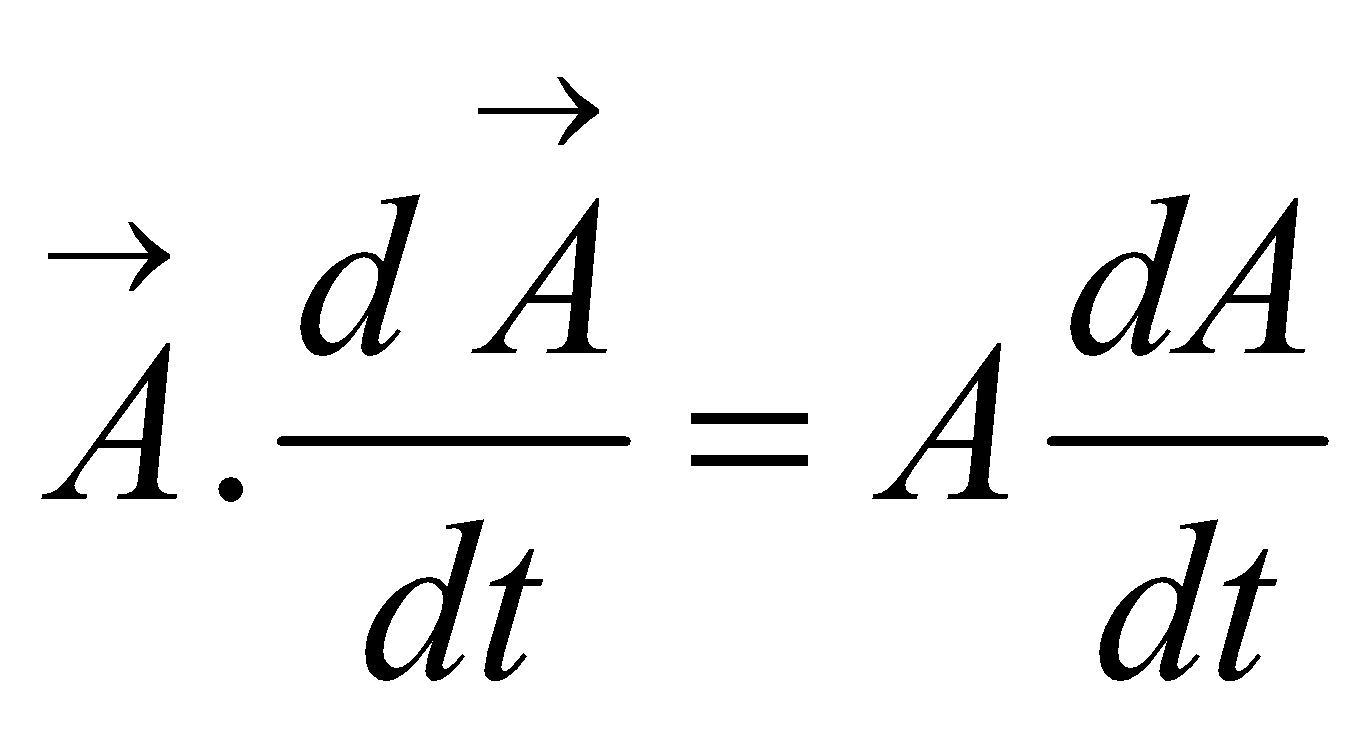Whereis the magnitude of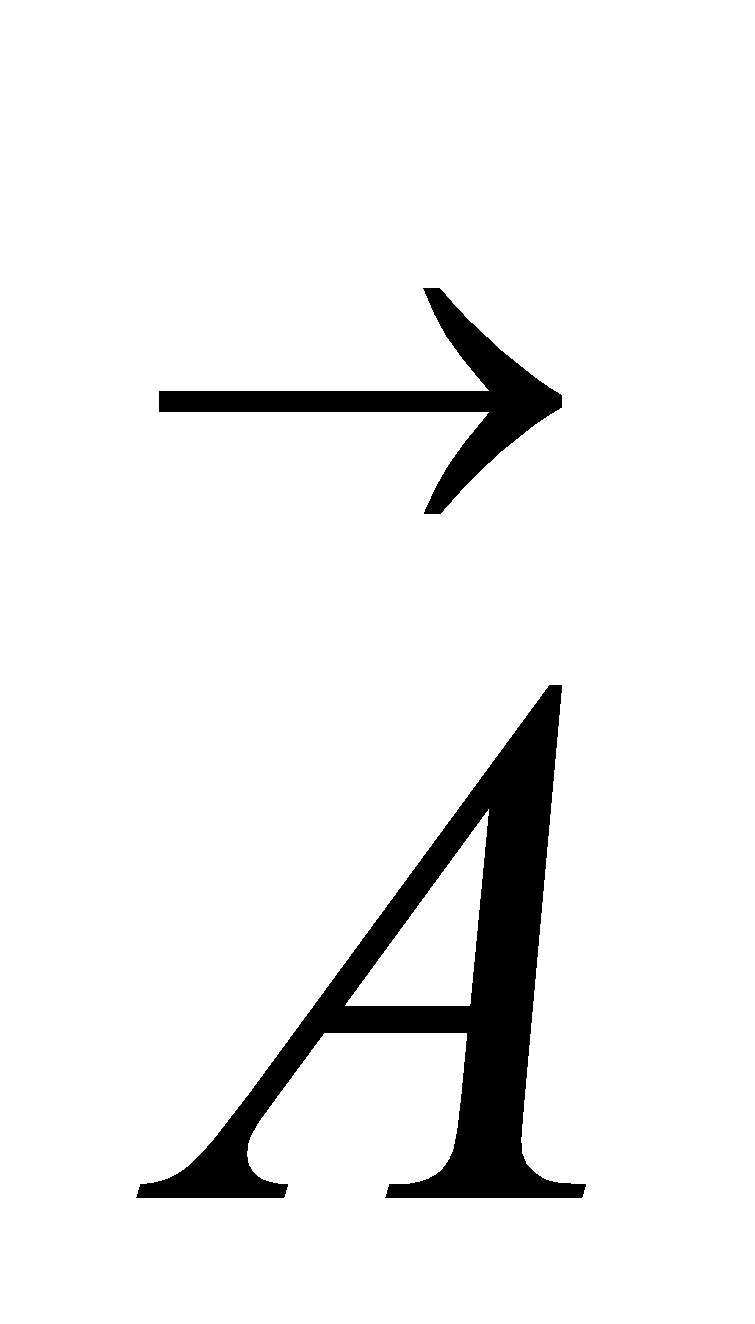. 2
10. (a) Show that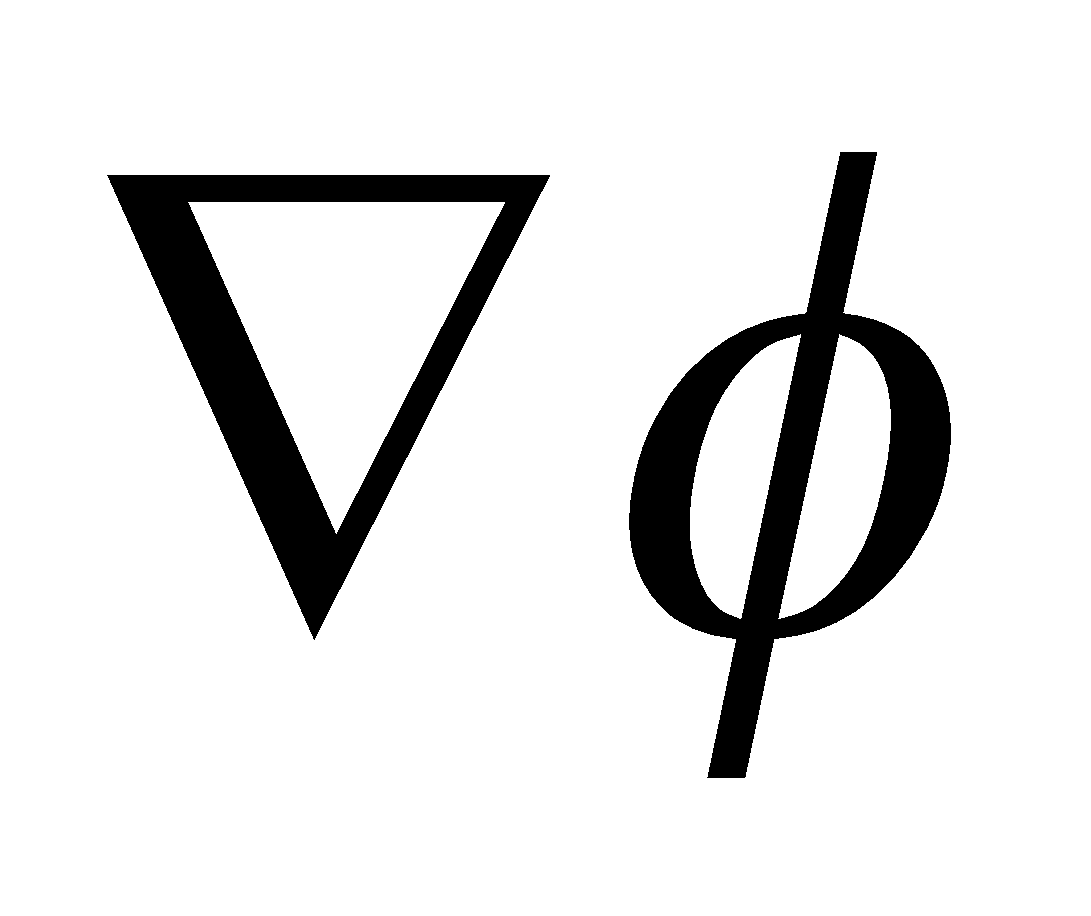is a vector perpendicular to the surface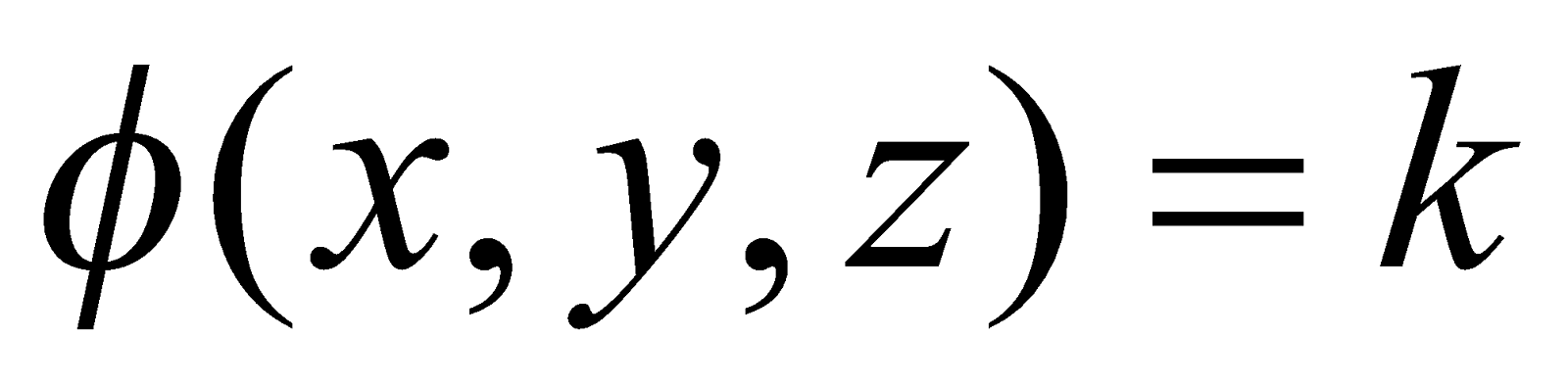, where K is a constant. 3
(b) Prove that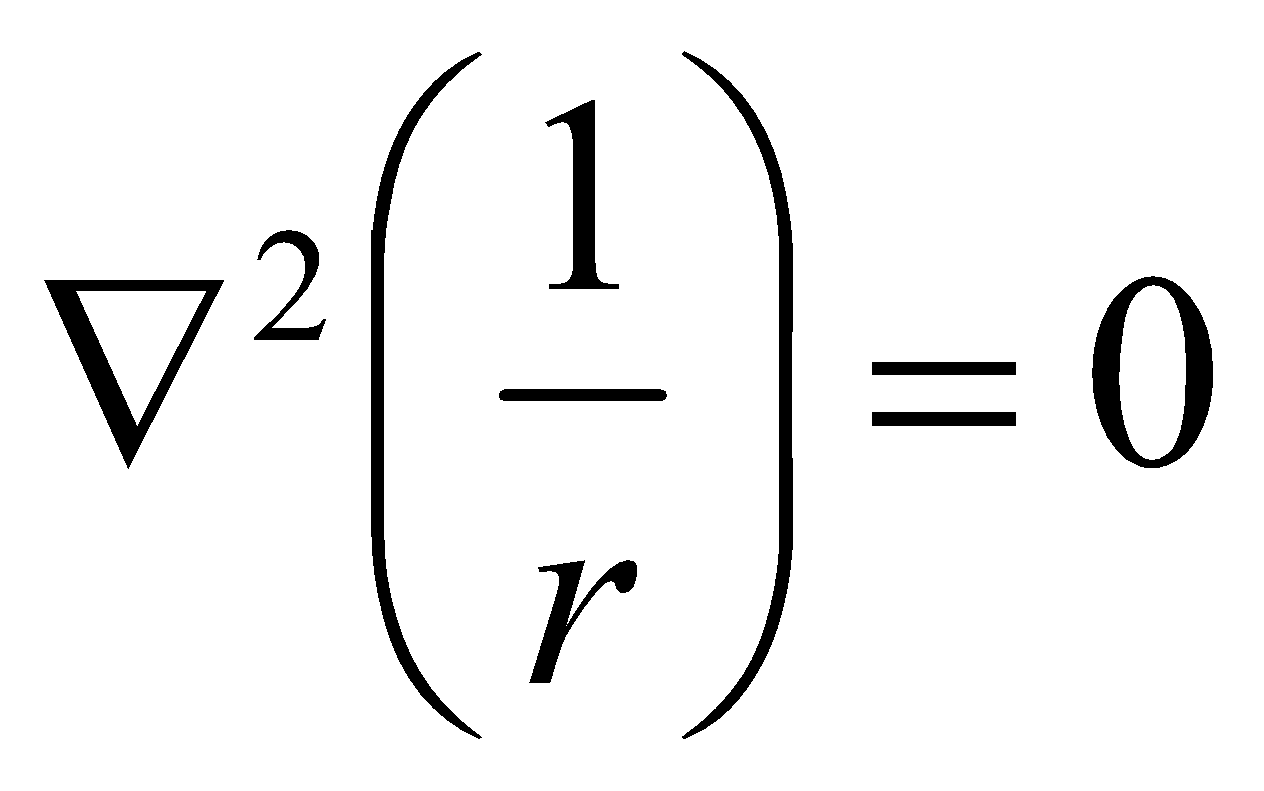4
(c) If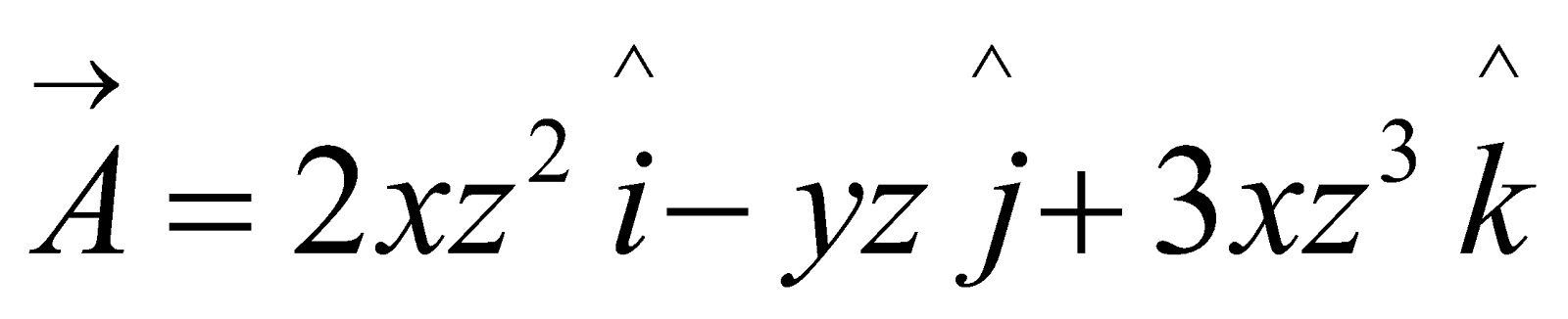and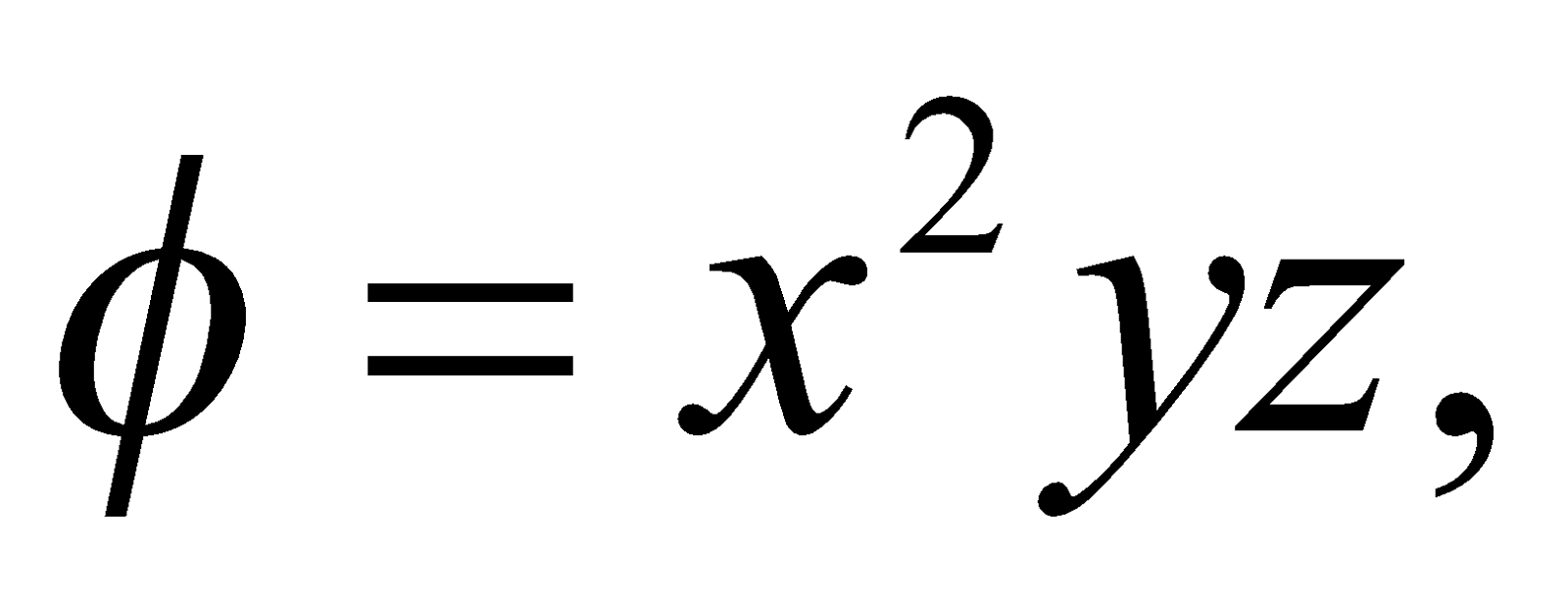then find curl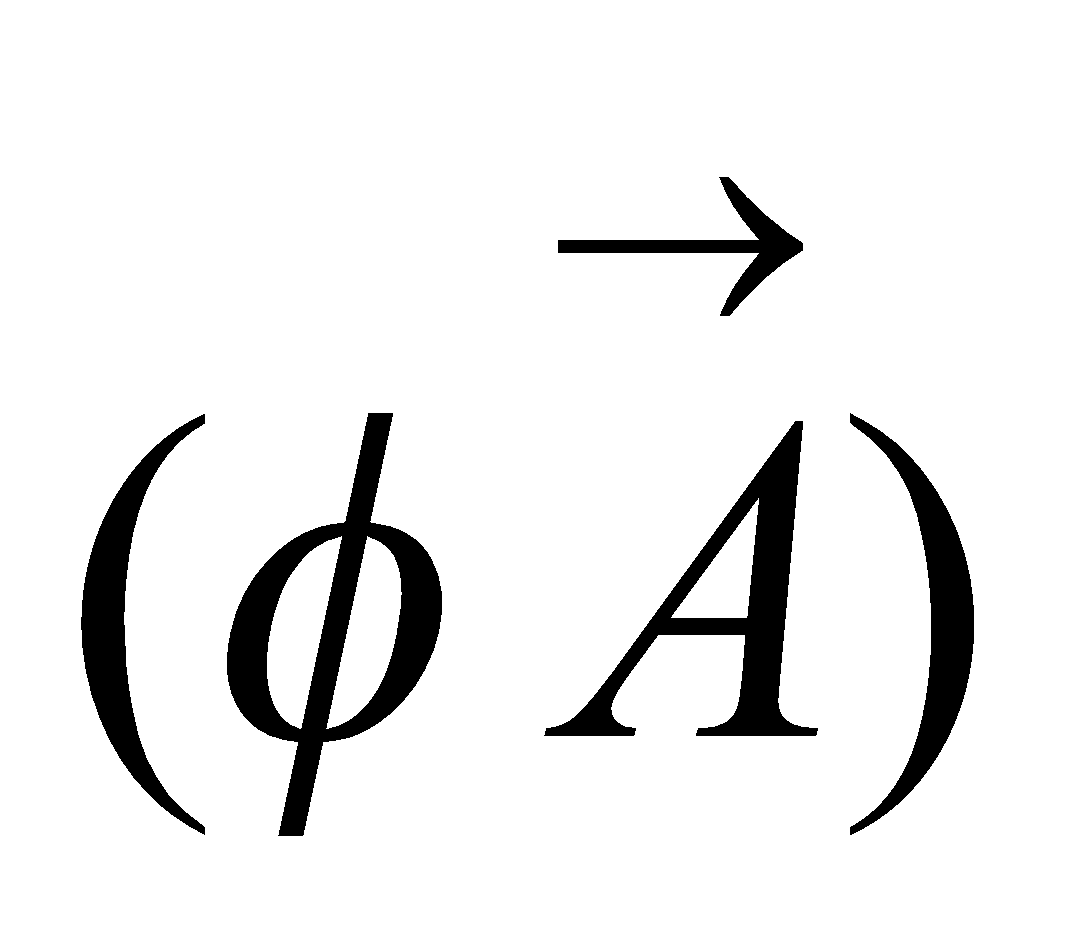at the point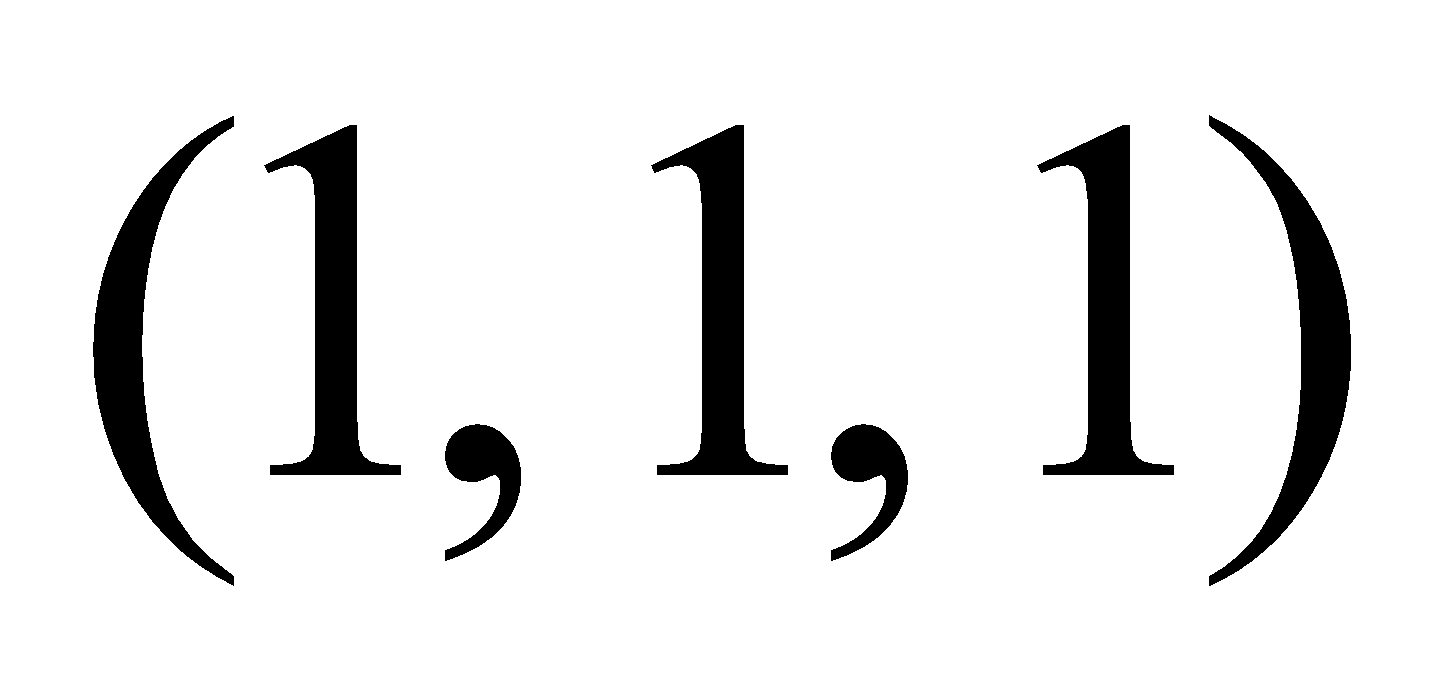. 4
Or
Prove that